# Basics of cubic spline models

## 2021-11-01

suppressMessages(suppressWarnings(library(broom)))
suppressMessages(suppressWarnings(library(dplyr)))
suppressMessages(suppressWarnings(library(Hmisc)))
suppressMessages(suppressWarnings(library(knitr)))
suppressMessages(suppressWarnings(library(magrittr)))
suppressMessages(suppressWarnings(library(rms)))
suppressMessages(suppressWarnings(library(splines)))
suppressMessages(suppressWarnings(library(tidyr)))

### Artificial data

Suppose you have some data where you suspect the behavior differs for x=1 to 5, x=6 to 10, x=11 to 15, and x=16 to 20.

library(ggplot2)
x <- 0:20
y <- rep(40, 21)
y[6:21] <- y[6:21]-3*(0:15)
y[11:21] <- y[11:21]+7*(0:10)
y[16:21] <- y[16:21]-(0:5)^2
y <- y+round(rnorm(21), 1)
simulated_example <- data.frame(x, y)
simulated_example
##     x    y
## 1   0 38.2
## 2   1 38.9
## 3   2 40.8
## 4   3 37.9
## 5   4 41.6
## 6   5 41.8
## 7   6 36.8
## 8   7 34.2
## 9   8 30.1
## 10  9 26.8
## 11 10 24.2
## 12 11 31.0
## 13 12 33.4
## 14 13 36.3
## 15 14 39.0
## 16 15 45.2
## 17 16 48.3
## 18 17 49.2
## 19 18 47.4
## 20 19 44.2
## 21 20 41.8
ggplot(simulated_example, aes(x, y)) +
geom_point()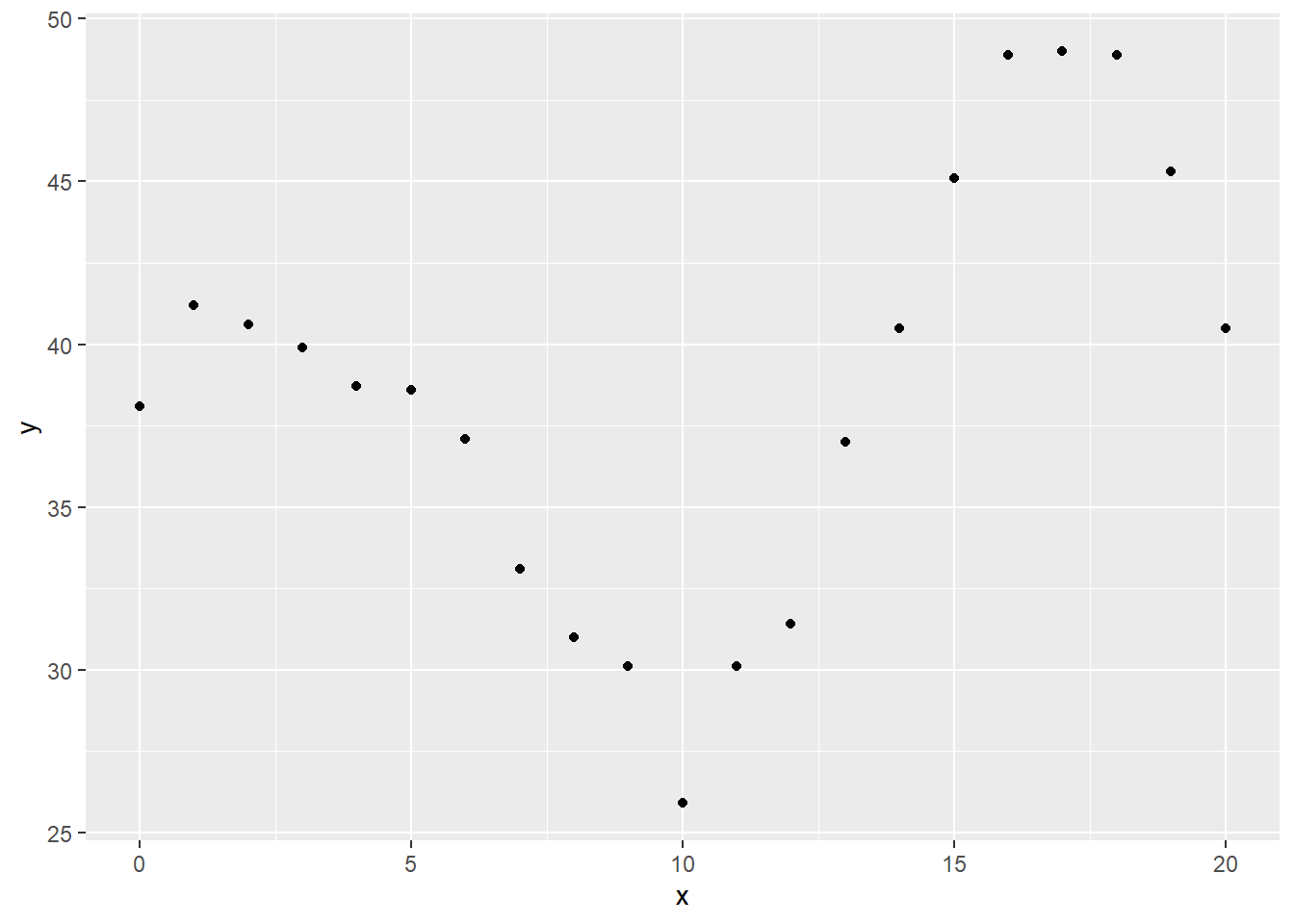To make the graphs look nice, you need to include some intermediate values.

x <- c(0:20, setdiff(seq(0, 20, by=1/64), 0:20))

You can get a variety of splines by defining constant, linear, quadratic, and cubic terms and then shift those functions to the right. After shifting, fill in the hole to the left with zeros.

xm <- data.frame(
c1 =rep(1, length(x)),
c2 =x,
c3 =x^2,
c4 =x^3,
c5 =(x> 5)*rep(1, length(x)),
c6 =(x> 5)*(x- 5),
c7 =(x> 5)*(x- 5)^2,
c8 =(x> 5)*(x- 5)^3,
c9 =(x>10)*rep(1, length(x)),
c10=(x>10)*(x-10),
c11=(x>10)*(x-10)^2,
c12=(x>10)*(x-10)^3,
c13=(x>15)*rep(1, length(x)),
c14=(x>15)*(x-15),
c15=(x>15)*(x-15)^2,
c16=(x>15)*(x-15)^3,
x=x,
y=NA
)
xm$y[1:21] <- y This is what the various pieces look like numerically … kable(xm[1:21, 1:16]) c1 c2 c3 c4 c5 c6 c7 c8 c9 c10 c11 c12 c13 c14 c15 c16 1 0 0 0 0 0 0 0 0 0 0 0 0 0 0 0 1 1 1 1 0 0 0 0 0 0 0 0 0 0 0 0 1 2 4 8 0 0 0 0 0 0 0 0 0 0 0 0 1 3 9 27 0 0 0 0 0 0 0 0 0 0 0 0 1 4 16 64 0 0 0 0 0 0 0 0 0 0 0 0 1 5 25 125 0 0 0 0 0 0 0 0 0 0 0 0 1 6 36 216 1 1 1 1 0 0 0 0 0 0 0 0 1 7 49 343 1 2 4 8 0 0 0 0 0 0 0 0 1 8 64 512 1 3 9 27 0 0 0 0 0 0 0 0 1 9 81 729 1 4 16 64 0 0 0 0 0 0 0 0 1 10 100 1000 1 5 25 125 0 0 0 0 0 0 0 0 1 11 121 1331 1 6 36 216 1 1 1 1 0 0 0 0 1 12 144 1728 1 7 49 343 1 2 4 8 0 0 0 0 1 13 169 2197 1 8 64 512 1 3 9 27 0 0 0 0 1 14 196 2744 1 9 81 729 1 4 16 64 0 0 0 0 1 15 225 3375 1 10 100 1000 1 5 25 125 0 0 0 0 1 16 256 4096 1 11 121 1331 1 6 36 216 1 1 1 1 1 17 289 4913 1 12 144 1728 1 7 49 343 1 2 4 8 1 18 324 5832 1 13 169 2197 1 8 64 512 1 3 9 27 1 19 361 6859 1 14 196 2744 1 9 81 729 1 4 16 64 1 20 400 8000 1 15 225 3375 1 10 100 1000 1 5 25 125 … and graphically. display_curve <- function(dat, lb) { # Plot a line connecting the points in dat # Assume that column 1 is x and column 2 is y # Work with either a data frame or matrix dat %>% data.frame %>% select(1:2) %>% set_names(c("x", "y")) %>% ggplot(aes(x, y)) + ggtitle(lb) + geom_line() + xlab(" ") + ylab(" ") } display_dots <- function(cplot, dat) { # Add dots in dat to an existing graph # Assume that column 1 is x and column 2 is y # Work with either a data frame or matrix dat %>% data.frame %>% set_names(c("x", "y")) -> df cplot + geom_point(data=df, aes(x, y)) } lb1 <- rep(c("Intercept", "Linear term", "Quadratic term", "Cubic term"), 4) lb2 <- rep(c("for the full range", "restarted at x=5", "restarted at x=10", "restarted at x=15"), each=4) lb <- paste(lb1, lb2) for (j in 1:16) { dat <- xm[ , c("x", paste0("c", j))] display_curve(dat, lb[j]) %>% display_dots(dat[1:21, ]) %>% plot }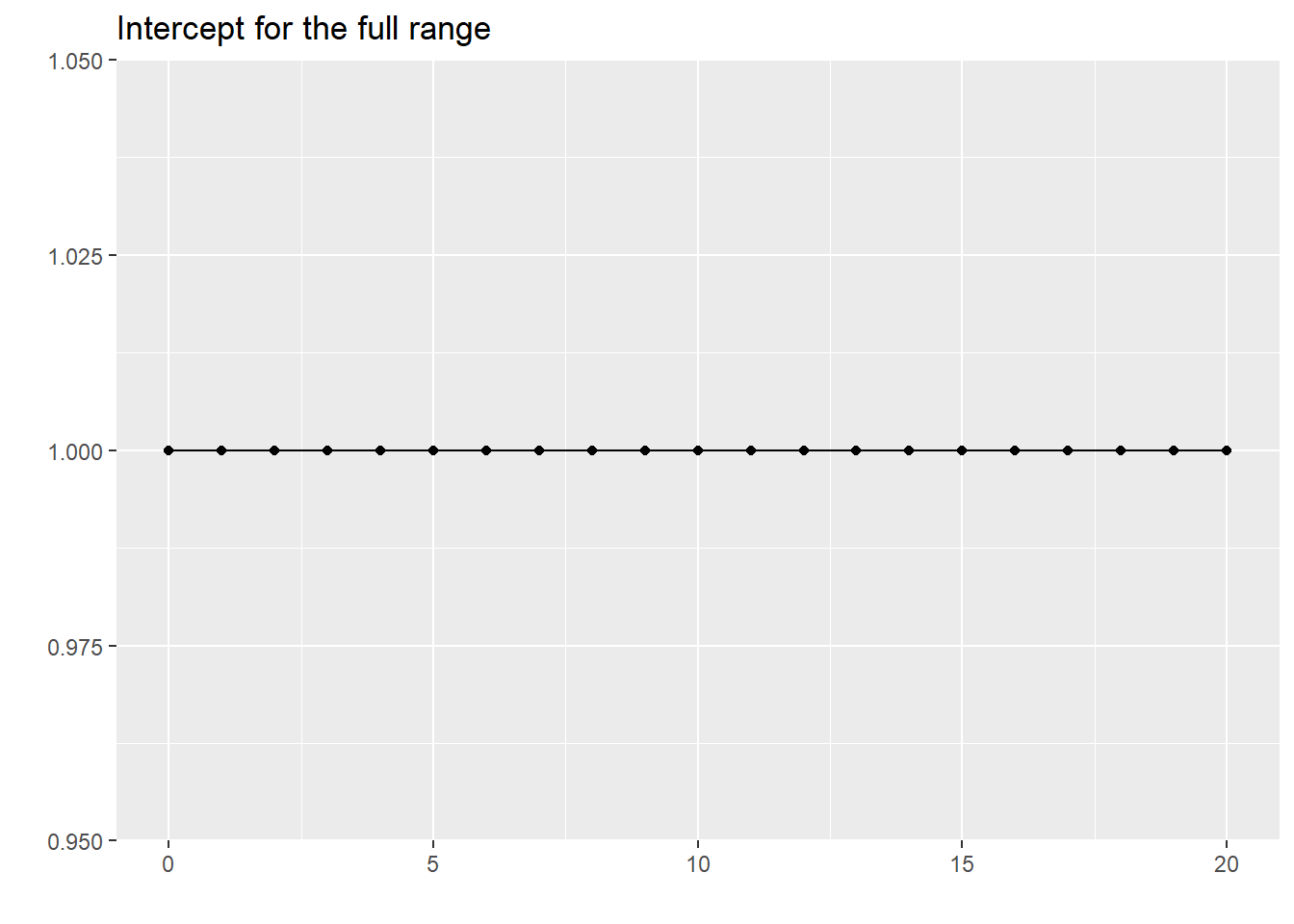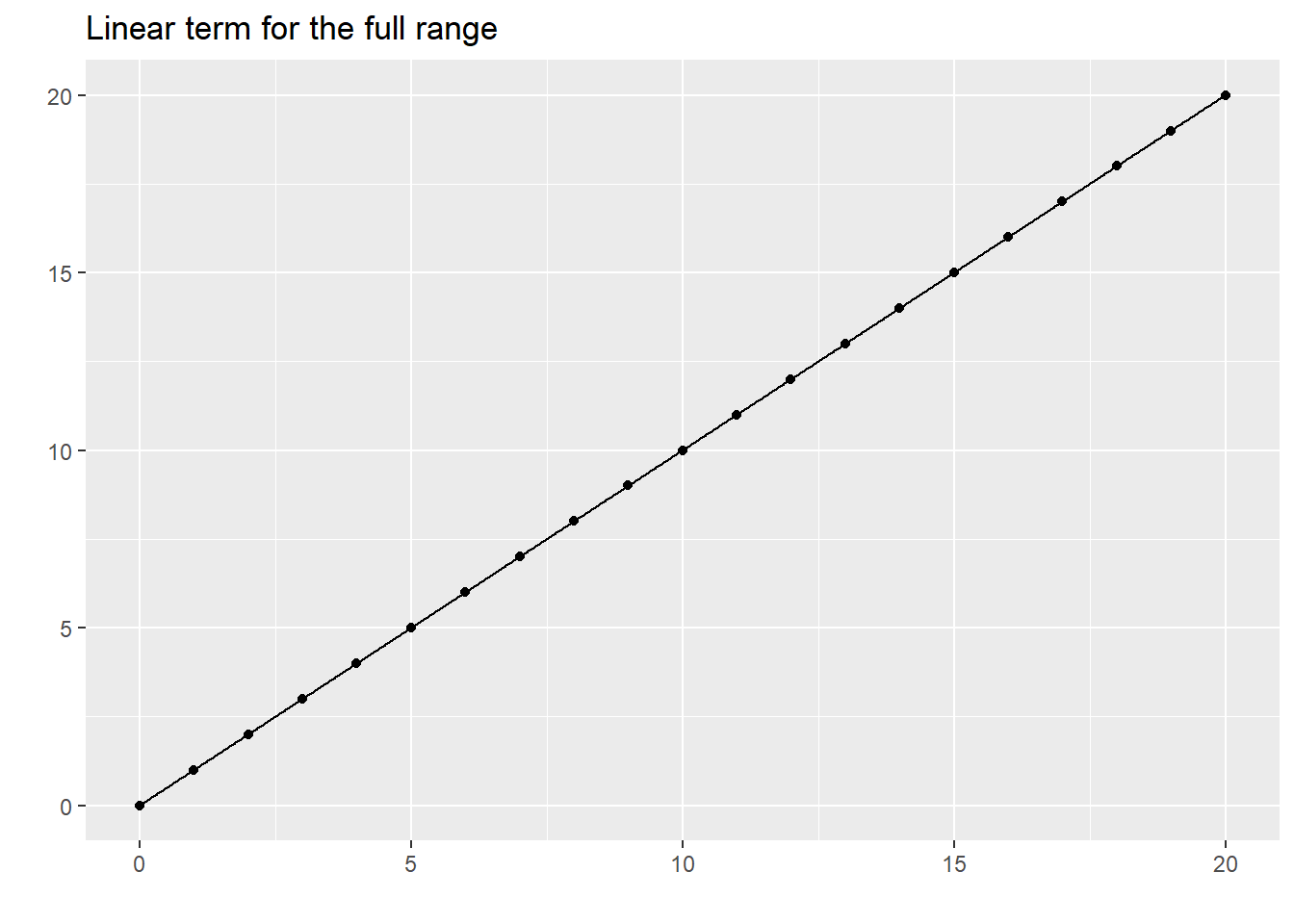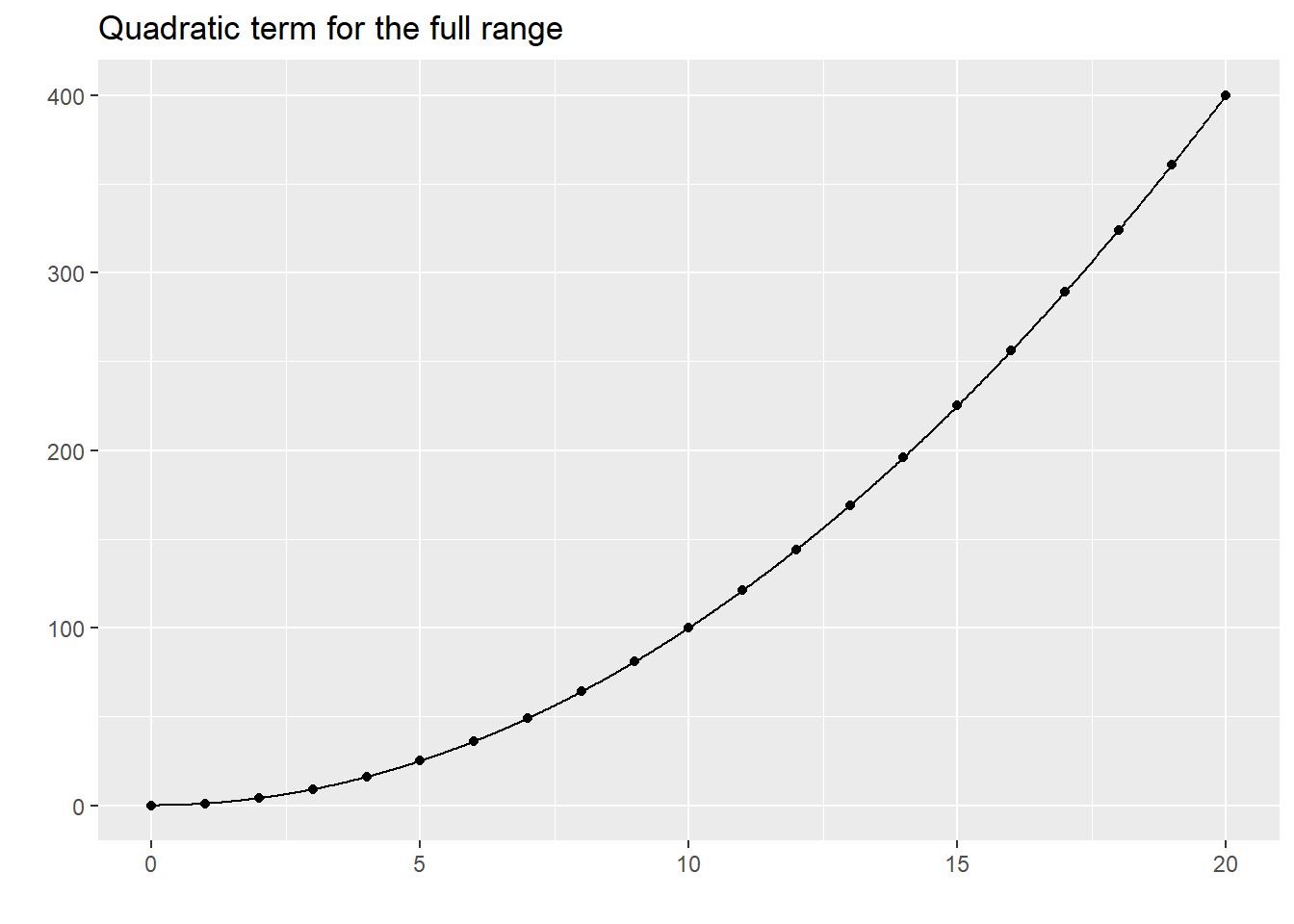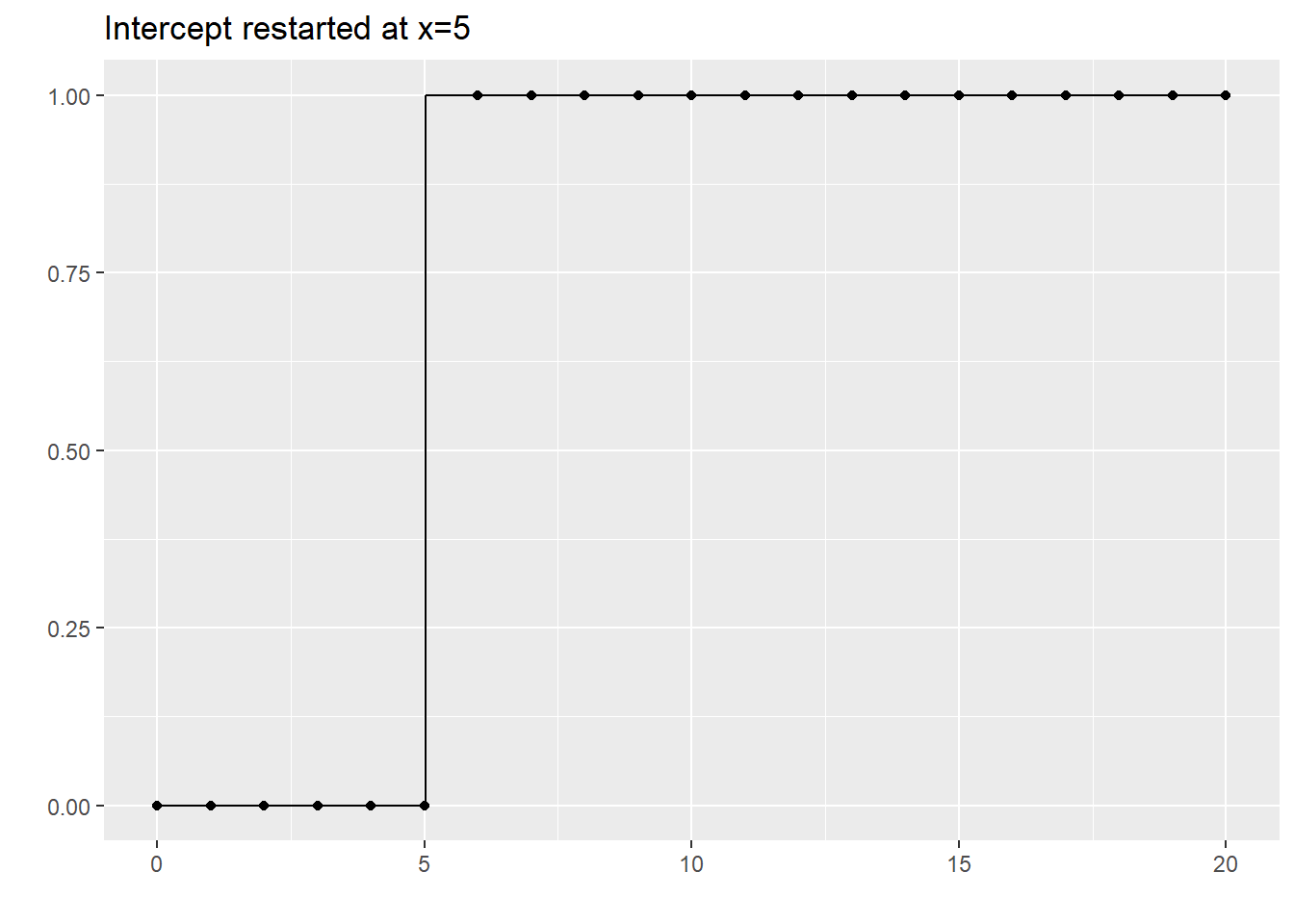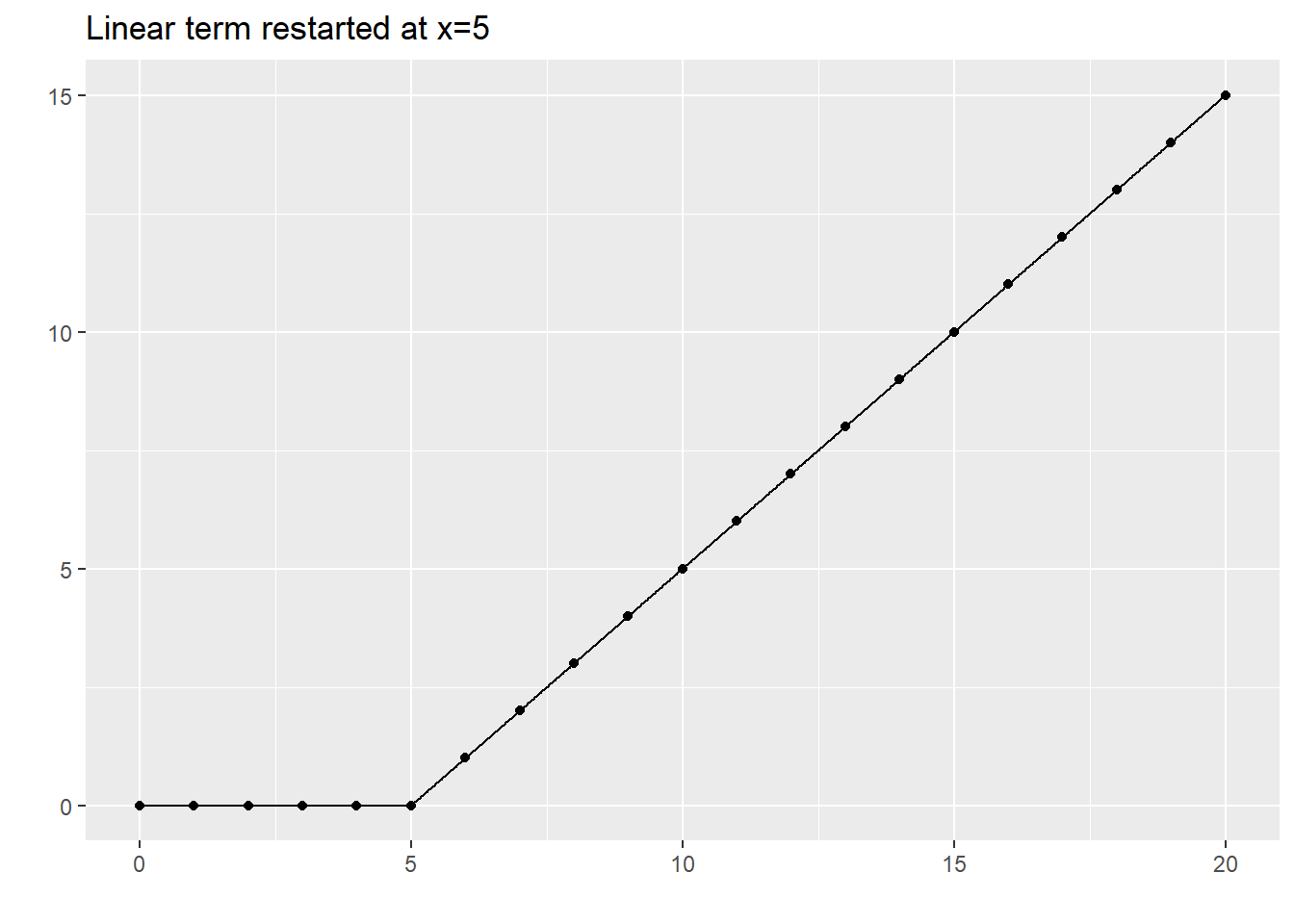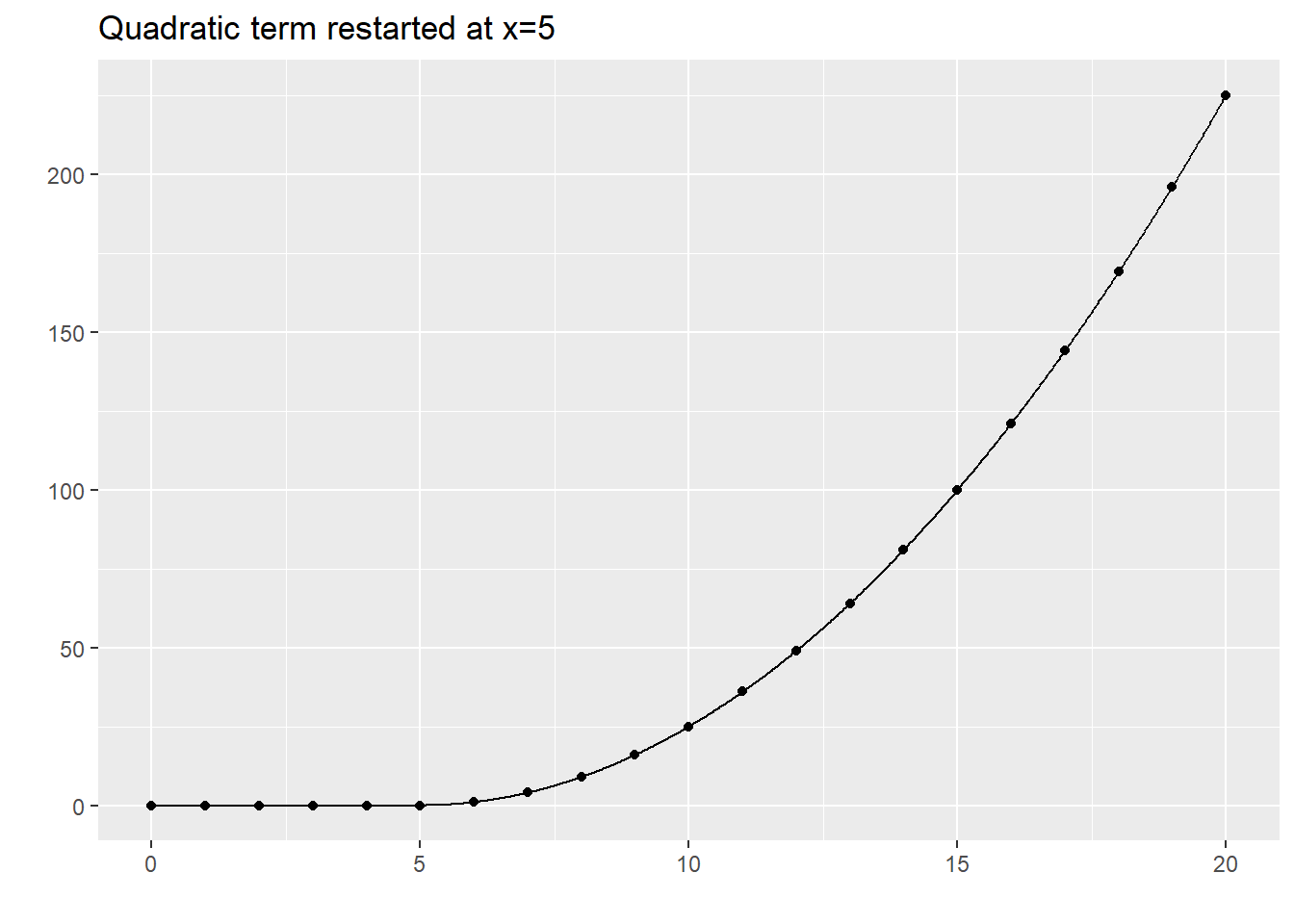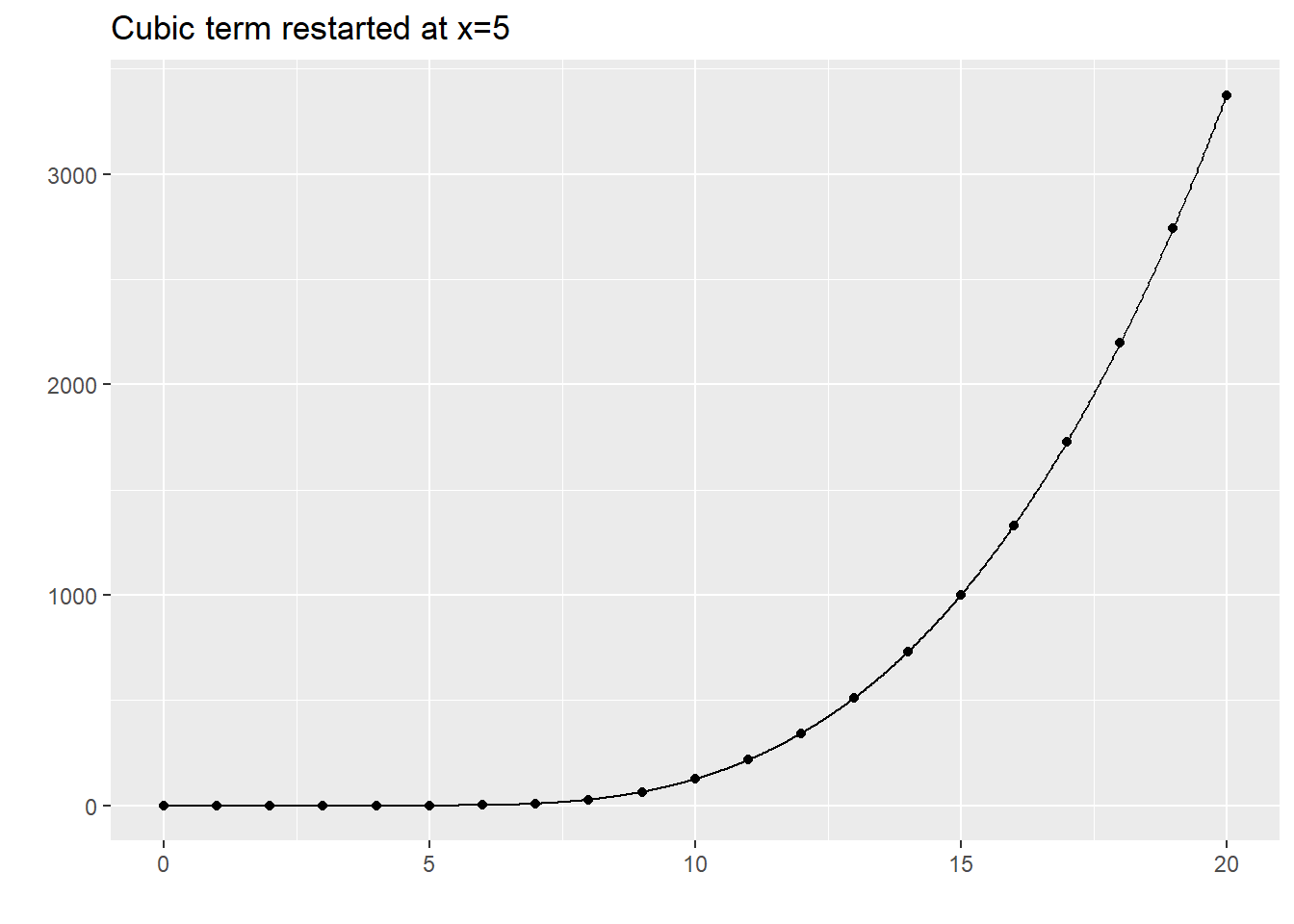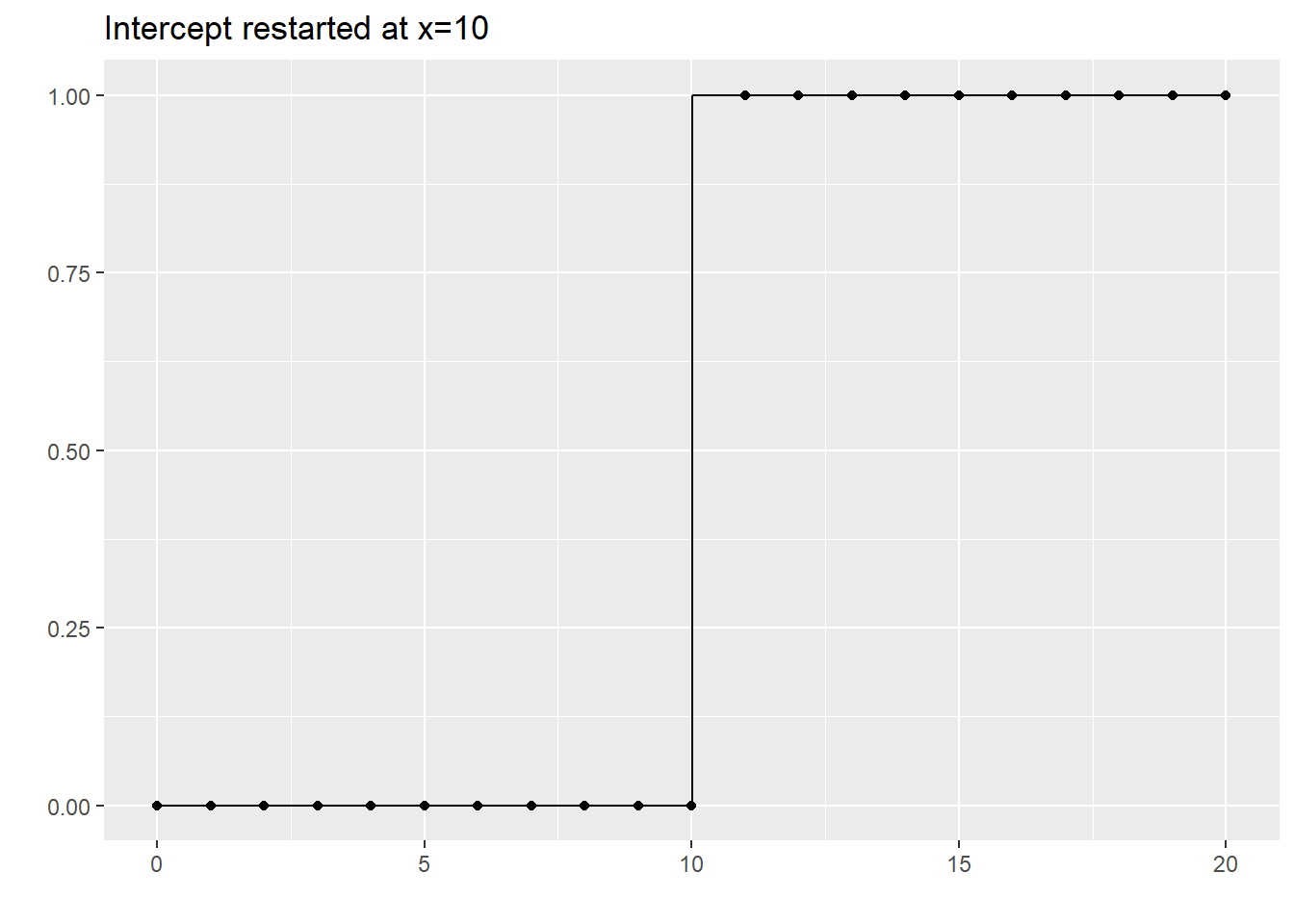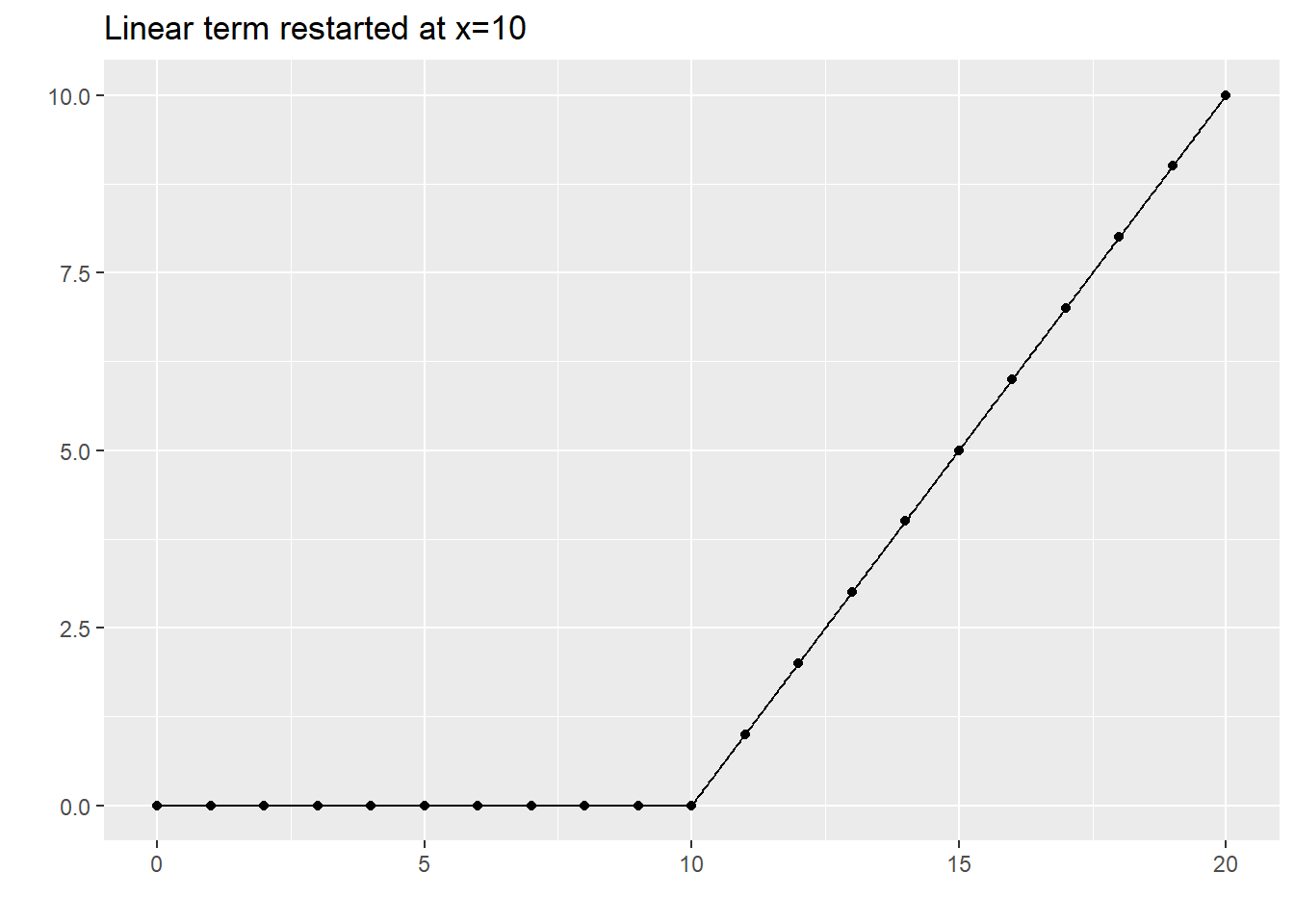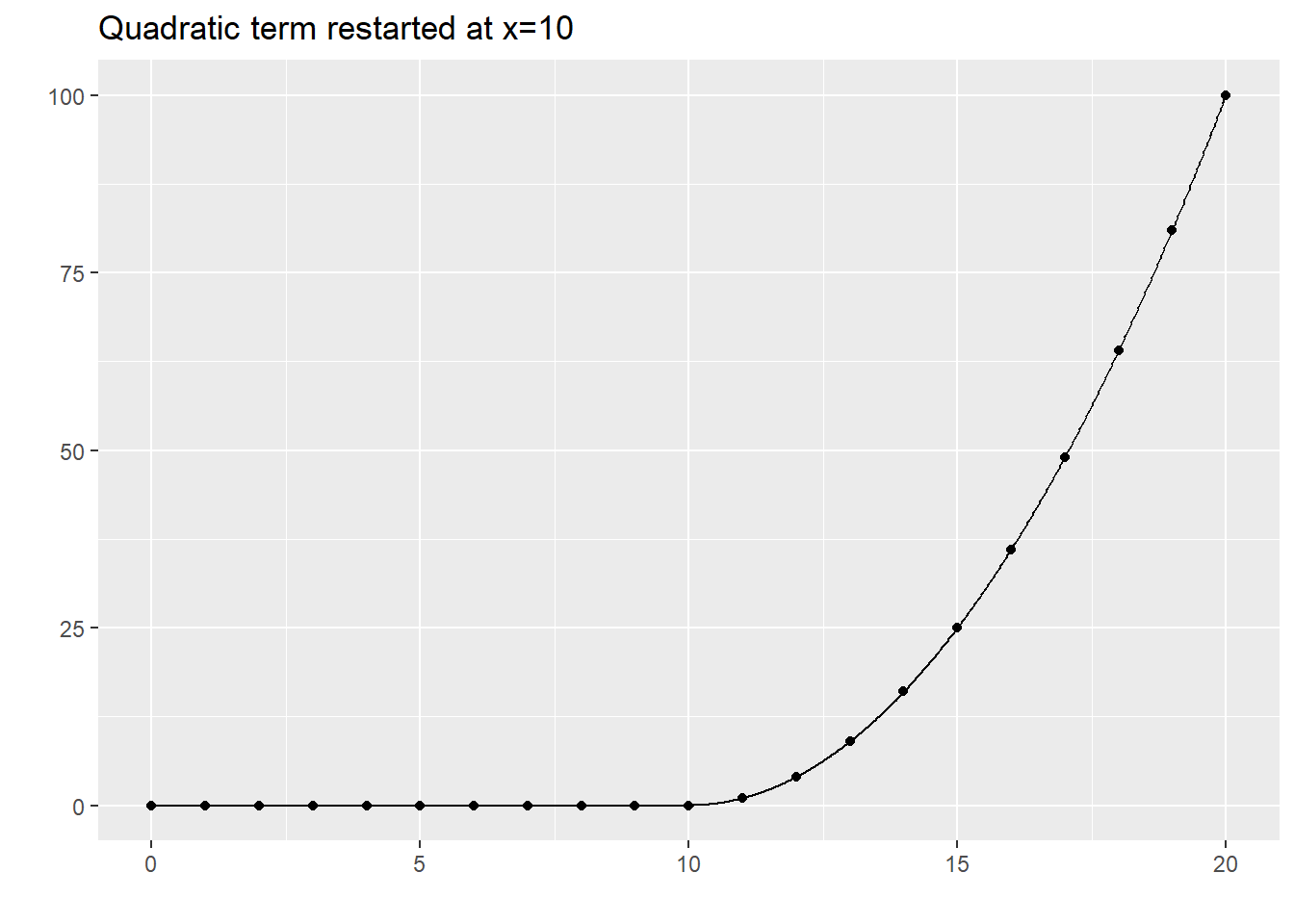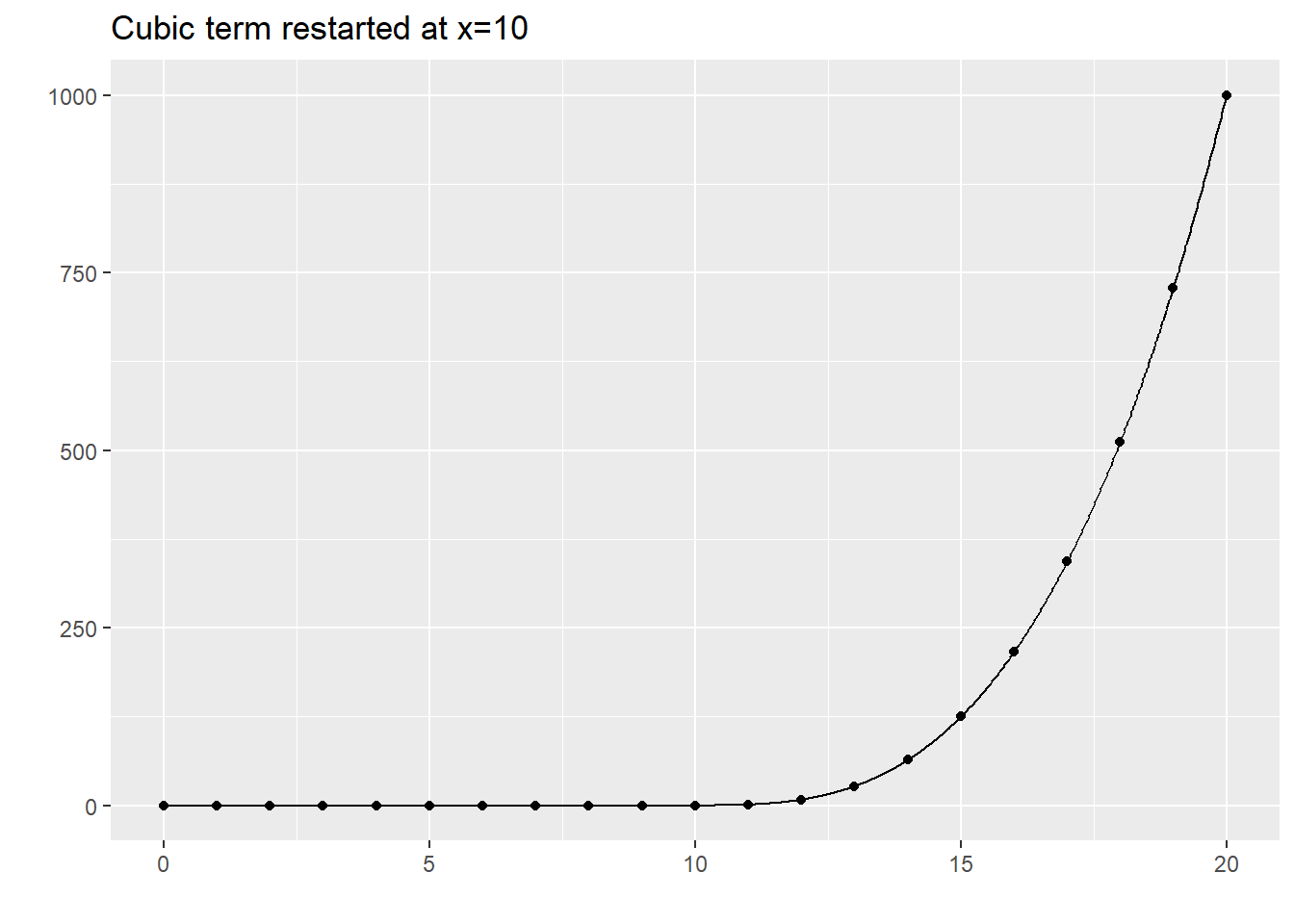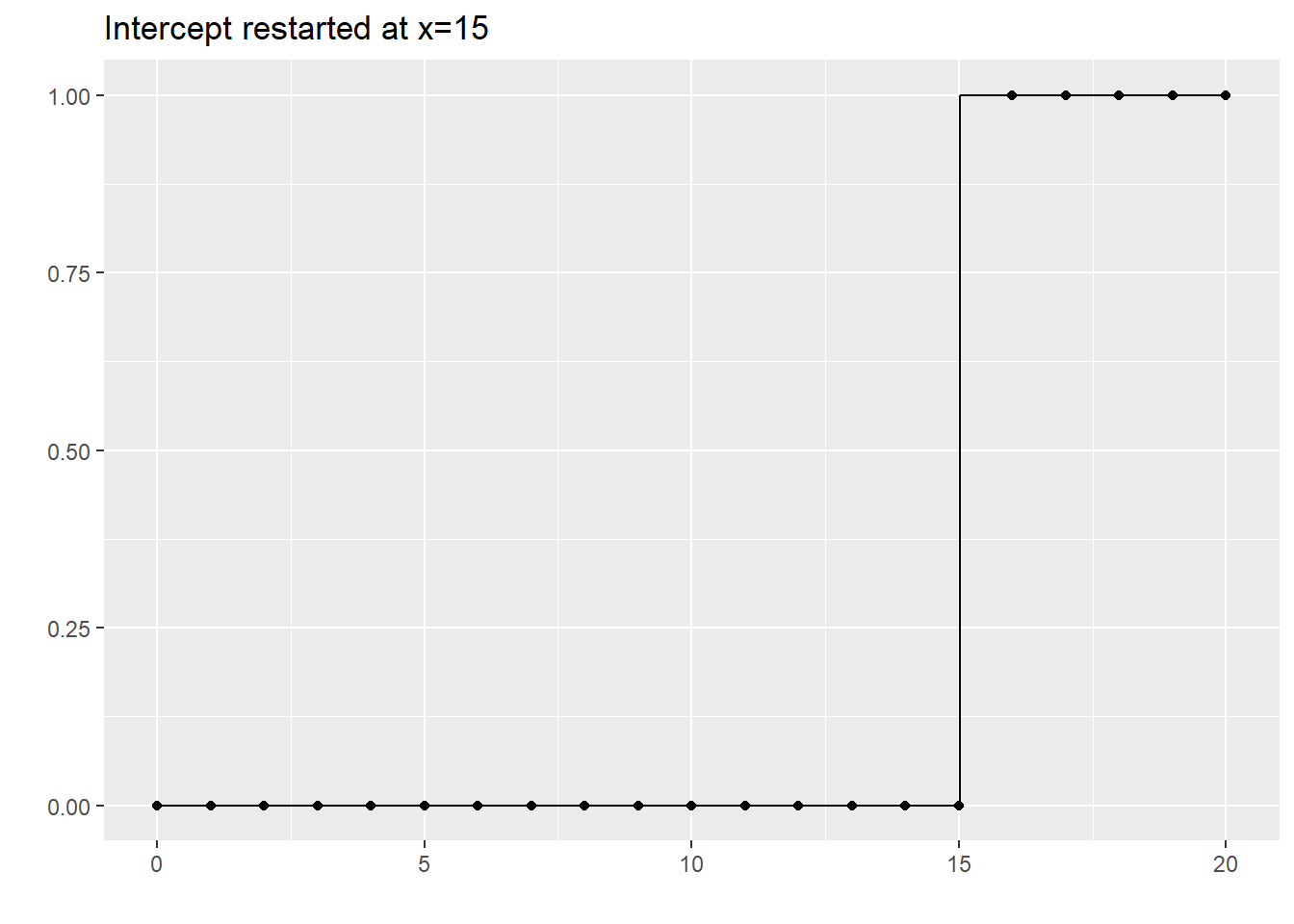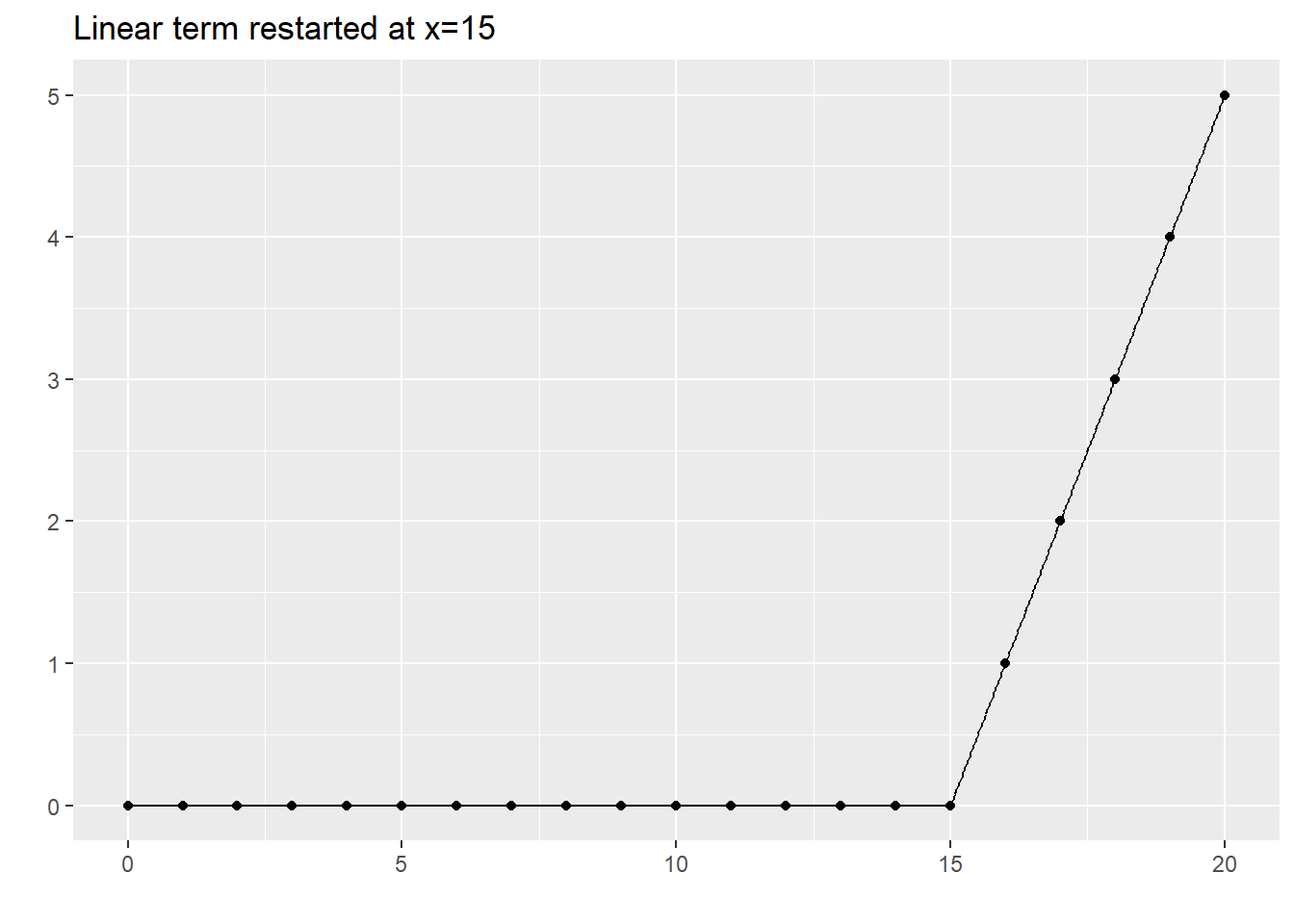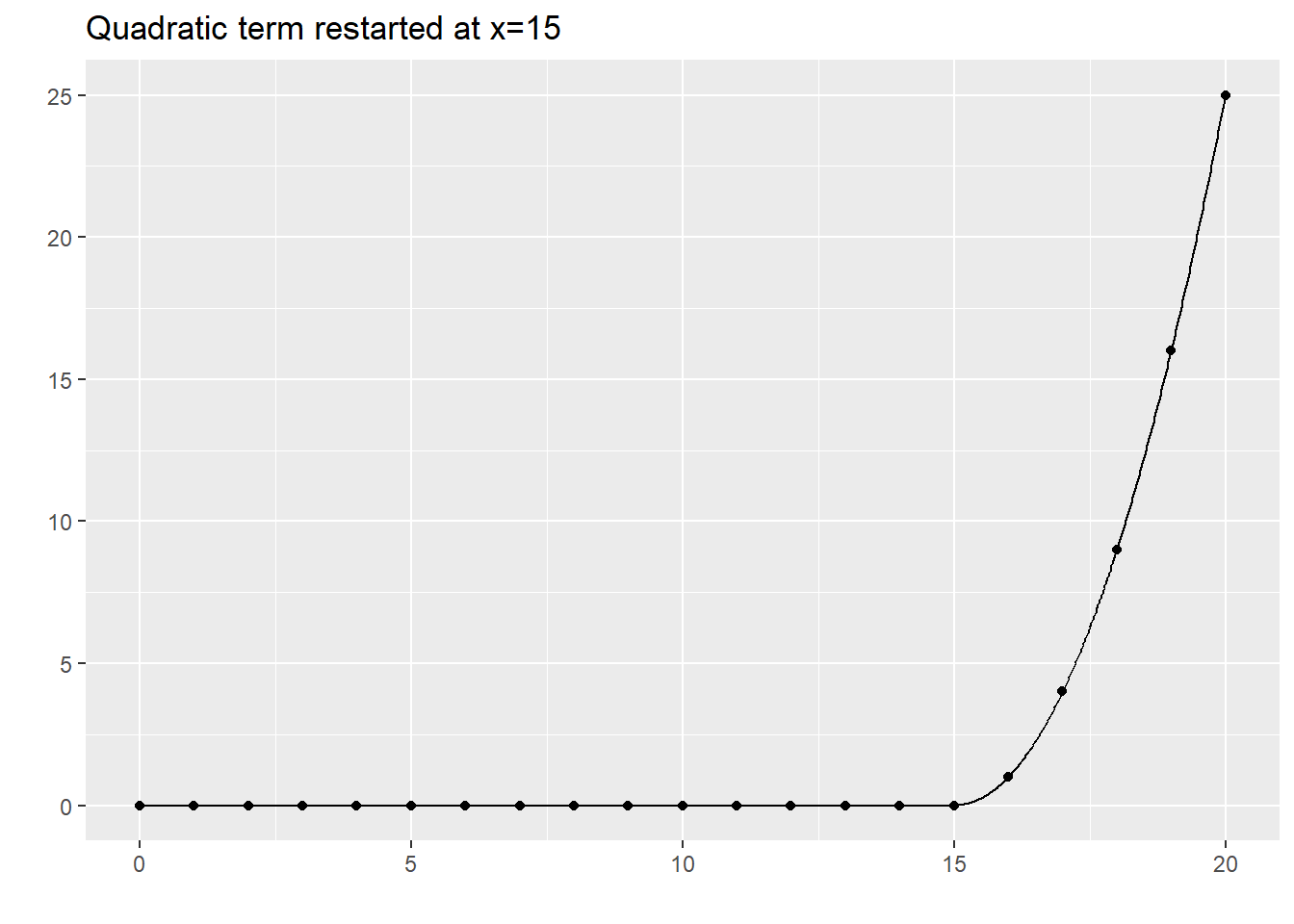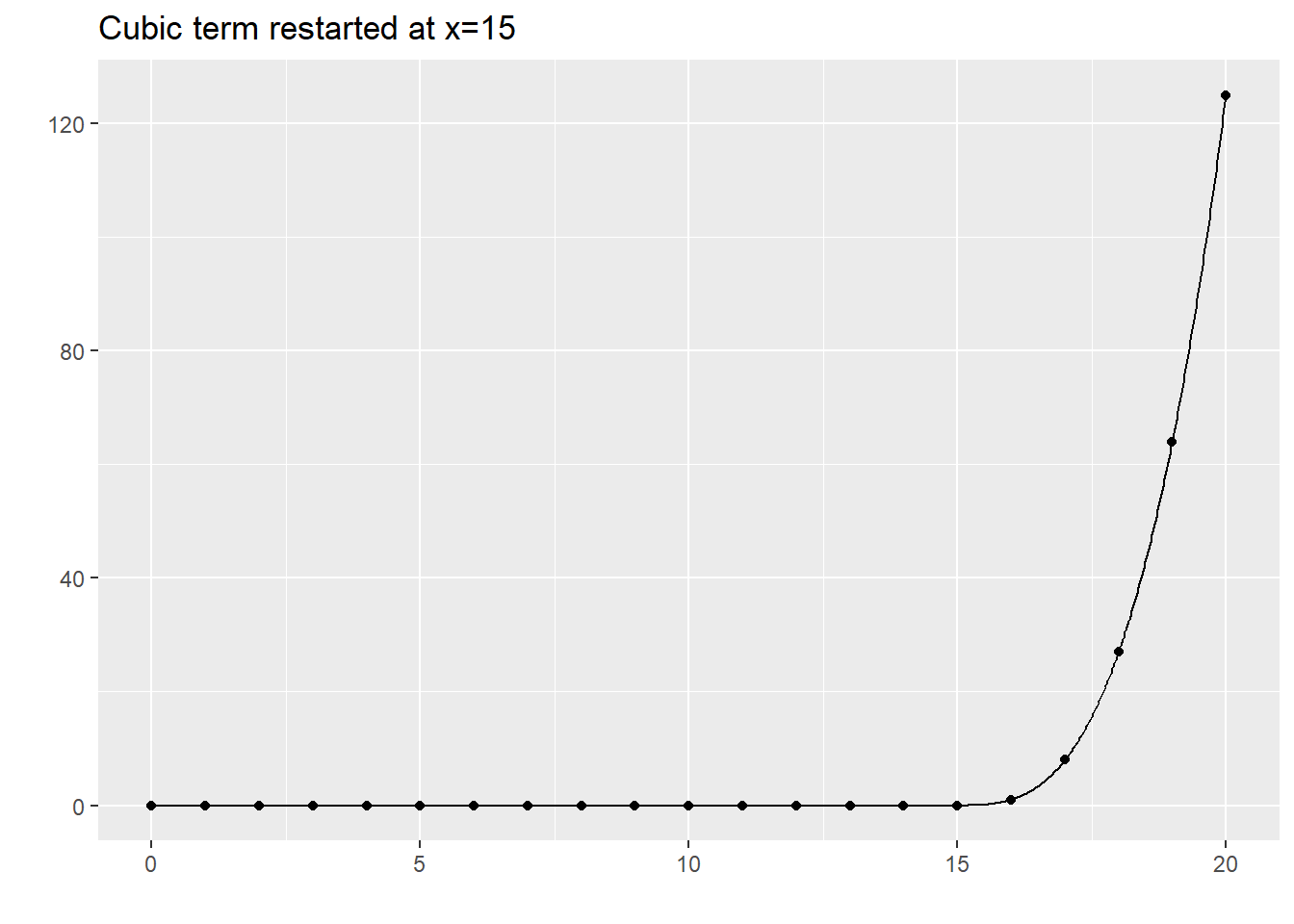### Single cubic polynomial The graph shown below represents the best fitting single cubic polynomial. lm(y~c2+c3+c4, data=xm) %>% augment(newdata=xm) %>% select(c2, .fitted) %>% display_curve("Single cubic fit") %>% display_dots(simulated_example)### Separate cubic polynomials So, we could fit a cubic model for the first five data points, for the second five, the third five, and the fourth five. This is a bit much: a cubic model has four parameters, so fitting four of them would use up 16 degrees of freedom in a data set with only 20 observations. But bear with me a bit on this. The trick to fitting four separate cubic polynomials is to “restart” the intercept, linear, quadratic, and cubic terms after x=5, x=10, and x=15, as shown above. This leads to a model with 16 degrees of freedom. This is way too many degrees of freedom for only 20 data points, but it helps anchor a series of more reasonable models. lm(y~c2+c3+c4+c5+c6+c7+c8+c9+c10+c11+c12+c13+c14+c15+c16, data=xm) %>% augment(newdata=xm) %>% select(c2, .fitted) %>% display_curve("Discontinous cubic splines") %>% display_dots(simulated_example)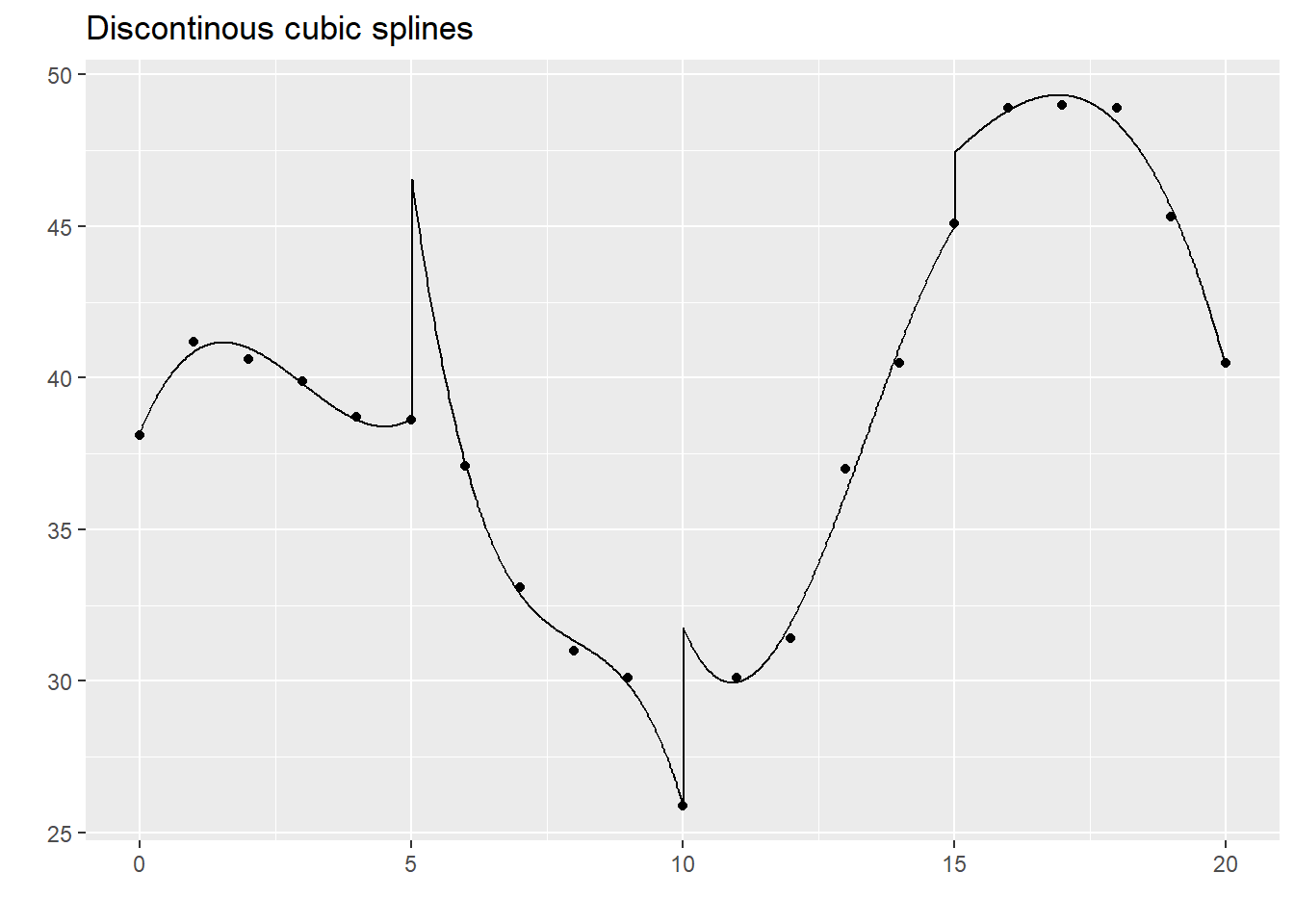This function is not continuous or smooth. To make the function continuous, drop the extra intercept terms. ### Continuous splines lm(y~c2+c3+c4+c6+c7+c8+c10+c11+c12+c14+c15+c16, data=xm) %>% augment(newdata=xm) %>% select(c2, .fitted) %>% display_curve("Continuous but not smooth cubic splines") %>% display_dots(simulated_example)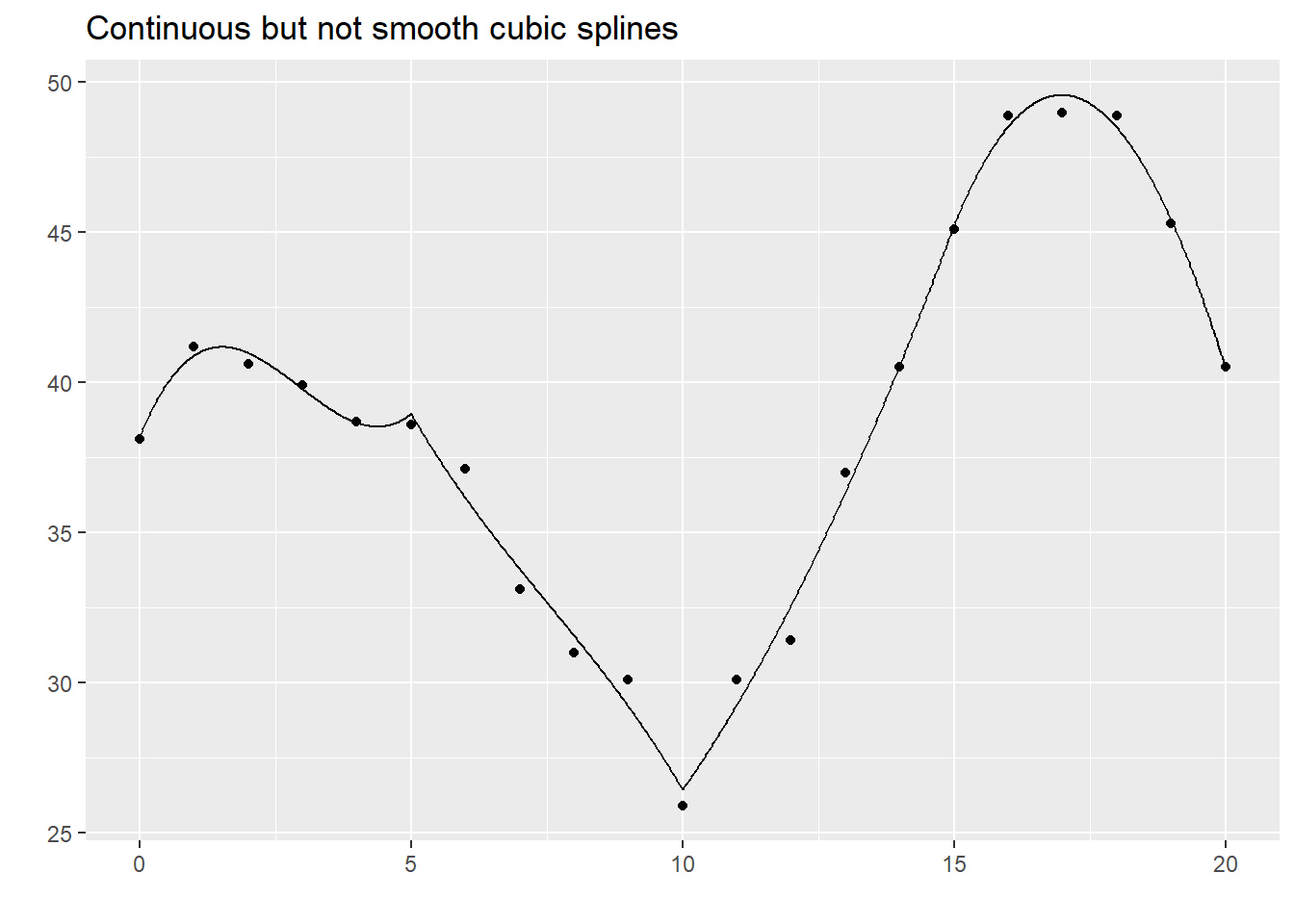While this graph is continuous, it still takes some abrupt turns. What this curve lacks is smoothness. The mathematical concept of smoothness is measured in terms of the continuity of derivatives. ### Smooth splines Here is a function that has a continuous first derivative. You fit this model by dropping the extra linear terms beyond the first one. Notice a pattern here. As you place additional restrictions on the spline (continuity, smoothness), you need fewer parameters. The four cubic models with no restrictions used up 16 degrees of freedom. When you added a continuity restriction, you only needed 13 degrees of freedom for the model. Add a smoothness restriction and you only need 10 degrees of freedom. lm(y~c2+c3+c4+c7+c8+c11+c12+c15+c16, data=xm) %>% augment(newdata=xm) %>% select(c2, .fitted) %>% display_curve("Continuous and smooth (1st derivative) cubic splines") %>% display_dots(simulated_example)Here is a function that has continuous first and second derivatives. This is a greater degree of smoothness than above and it requires only 7 degrees of freedom. lm(y~c2+c3+c4+c8+c12+c16, data=xm) %>% augment(newdata=xm) %>% select(c2, .fitted) %>% display_curve("Continous and smooth (1st and 2nd derivatives) cubic splines") %>% display_dots(simulated_example)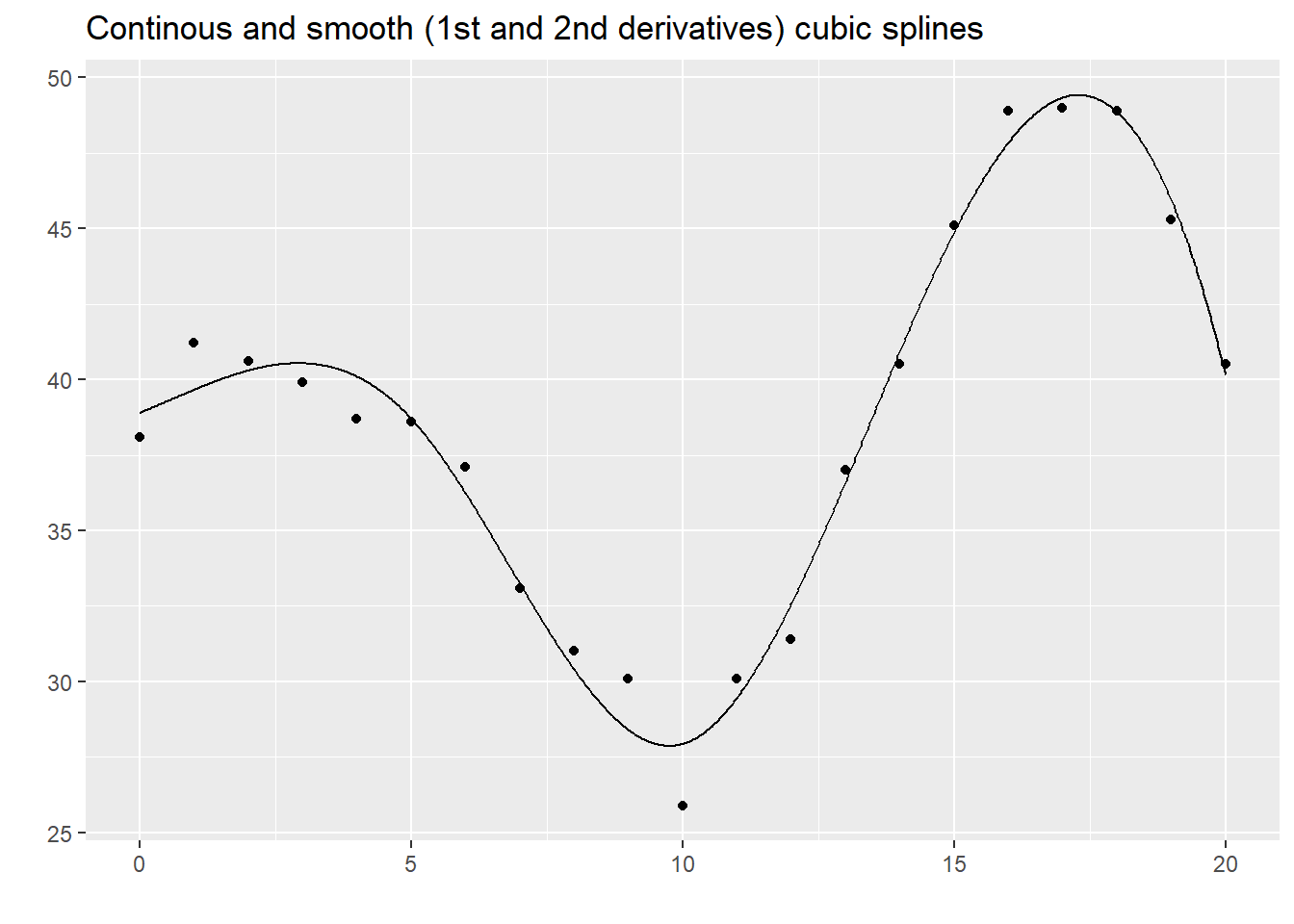This is what most people refer to when they talk about splines: a piecewise cubic model with continuity and continuous first and second derivatives. It is a fairly simple model (not that many degrees of freedom), but it produces a curve that has the flexibility to fit a variety of curves that have the aesthetically pleasing features of continuity and smoothness. Continuity and smoothness are more than just aesthetics, though. There are many scientific settings where we expect no jumps (discontinuities) and no abrupt turns (lack of smoothness). If you are measuring the onset of symptoms from a disease, you know that the viruses or bacteria that are causing the disease are increasing in a continuous and smooth pattern. So any problems that they cause should also increase in a continuous and smooth pattern. Other settings, however, should not necessarily be expected to produce continuous and smooth outcomes. If a particular metabolic pathway becomes saturated or an anotomical barrier is breached, the suddenness transition could result in an abrupt turn or a discontinuity. So do think about the particular context of your problem when deciding what type of spline model to use. This approach is simple and easy to follow, but there is one catch. There is an issue with multicollinearity. round(cor(xm[c(2:4, 8, 12, 16)]), 2) ## c2 c3 c4 c8 c12 c16 ## c2 1.00 0.97 0.92 0.85 0.73 0.55 ## c3 0.97 1.00 0.99 0.95 0.86 0.67 ## c4 0.92 0.99 1.00 0.99 0.93 0.76 ## c8 0.85 0.95 0.99 1.00 0.97 0.83 ## c12 0.73 0.86 0.93 0.97 1.00 0.92 ## c16 0.55 0.67 0.76 0.83 0.92 1.00 The correlations are quite high and this can lead to computational problems, including rounding errors. So most spline models implemented on a computer use a different approach. ### B-splines B-splines provide a solution with less issues of multi-collinearity. You use the bs function in the splines package to compute B-splines. cubic_spline <- bs(xm$c2, knots=c(5, 10, 15), degree=3, intercept=TRUE)

The individual columns represent piecwise cubic polynomials. Notice how they are concentrated in certain intervals and there is only a partial overlap between these intervals. Notice also how they transition smoothly to zero outside those intervals.

for (j in 1:dim(cubic_spline)) {
dat <- cbind(xm$c2, cubic_spline[ , j]) display_curve(dat, "Cubic B-spline terms") %>% display_dots(dat[1:21, ]) %>% plot }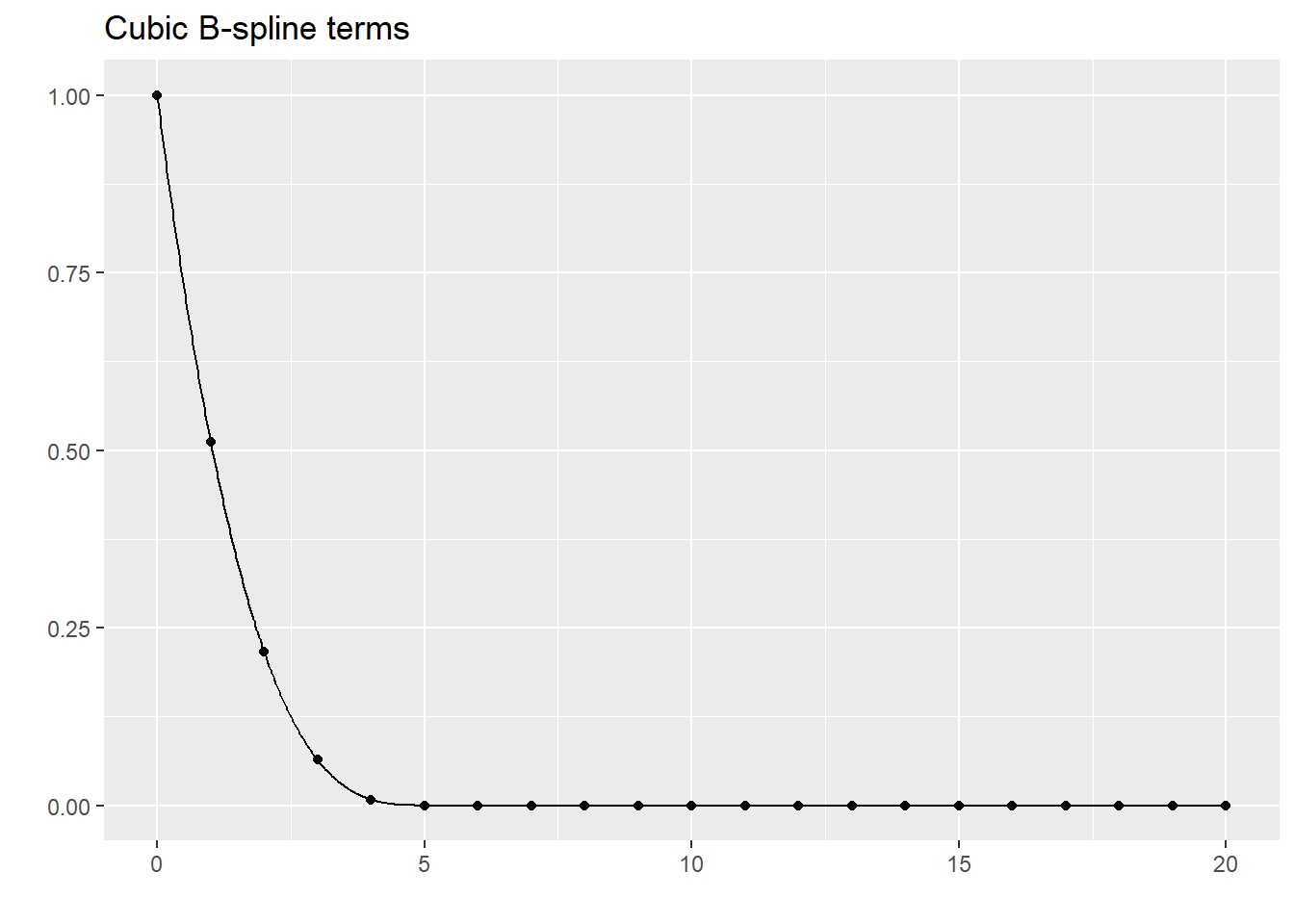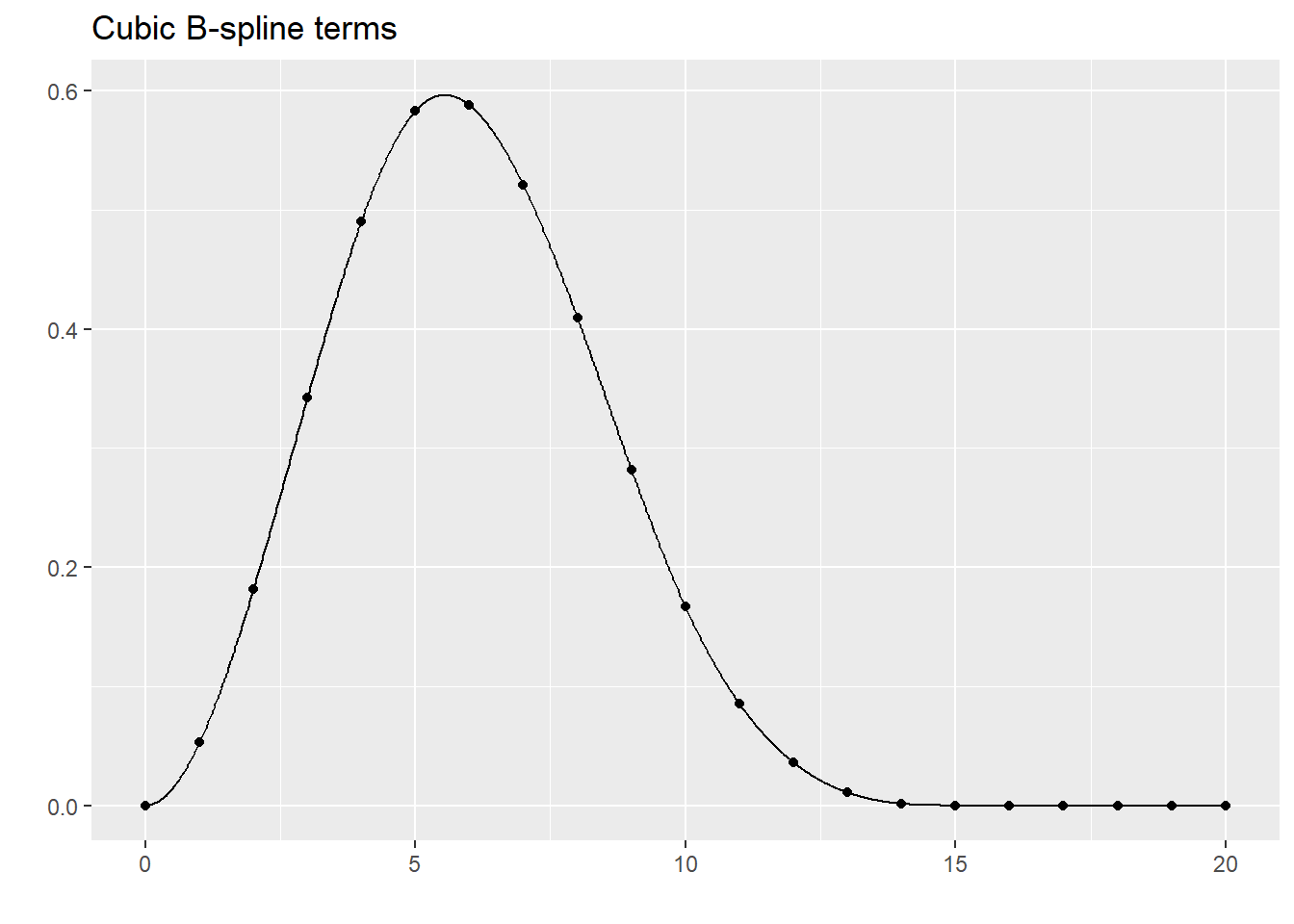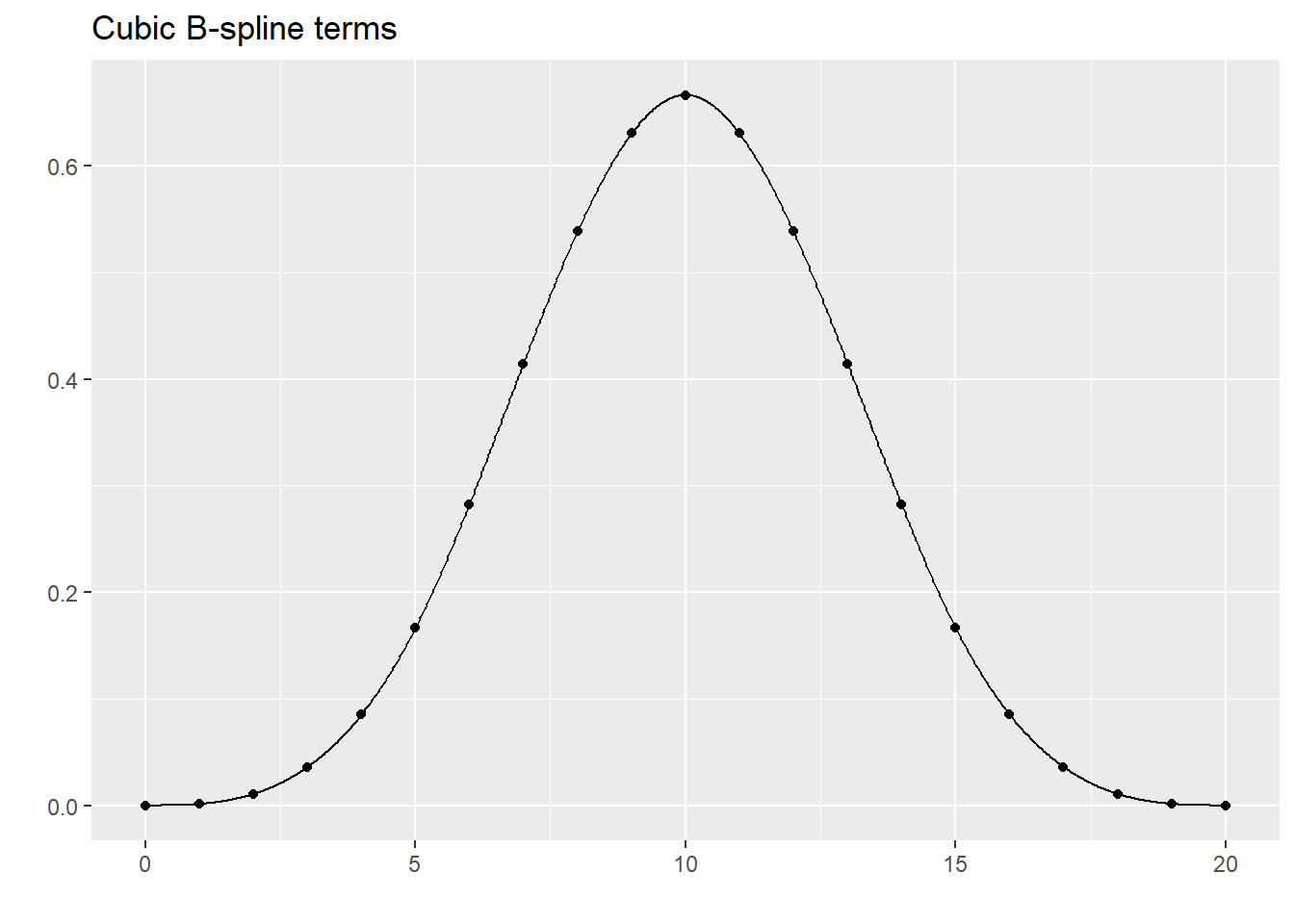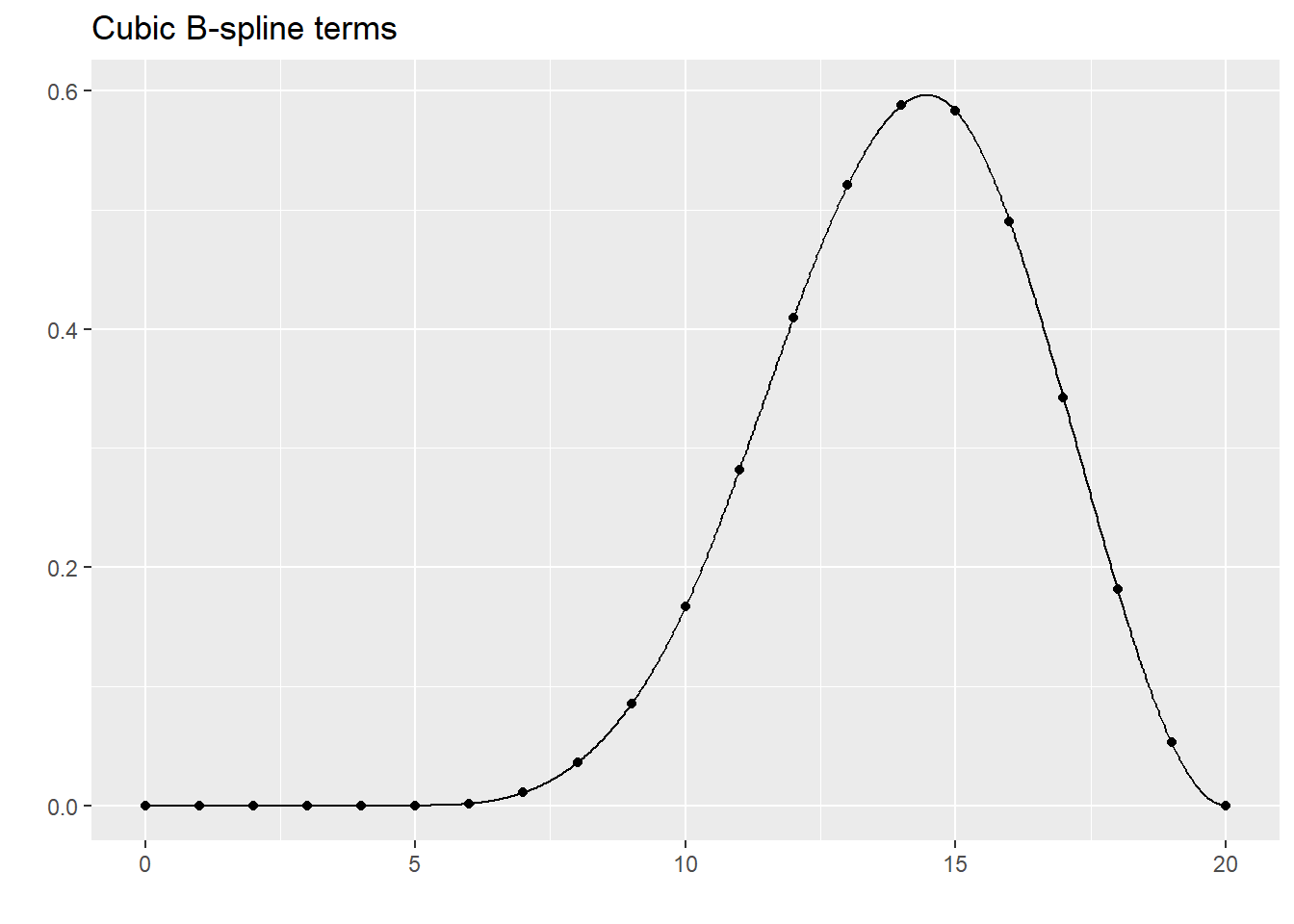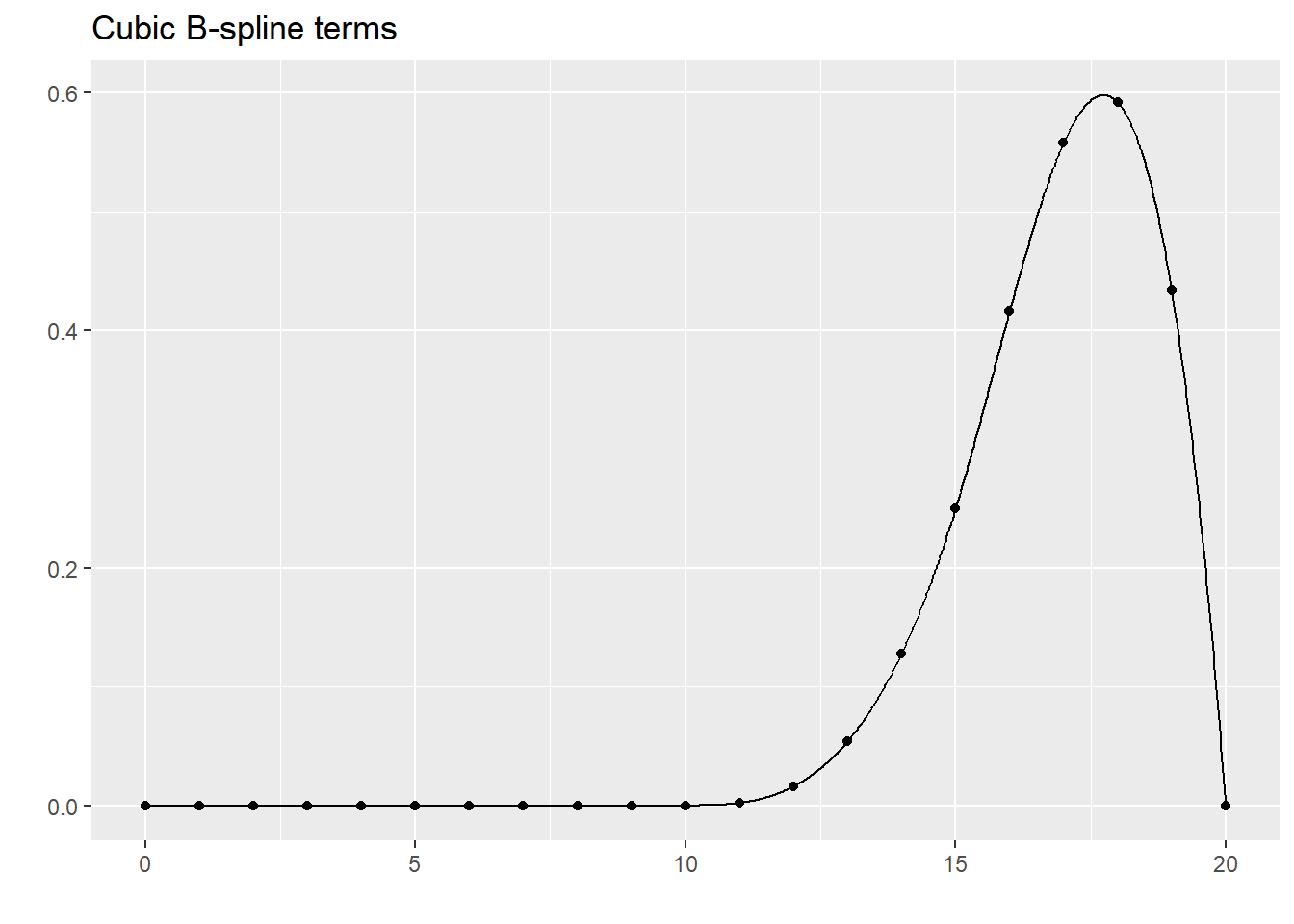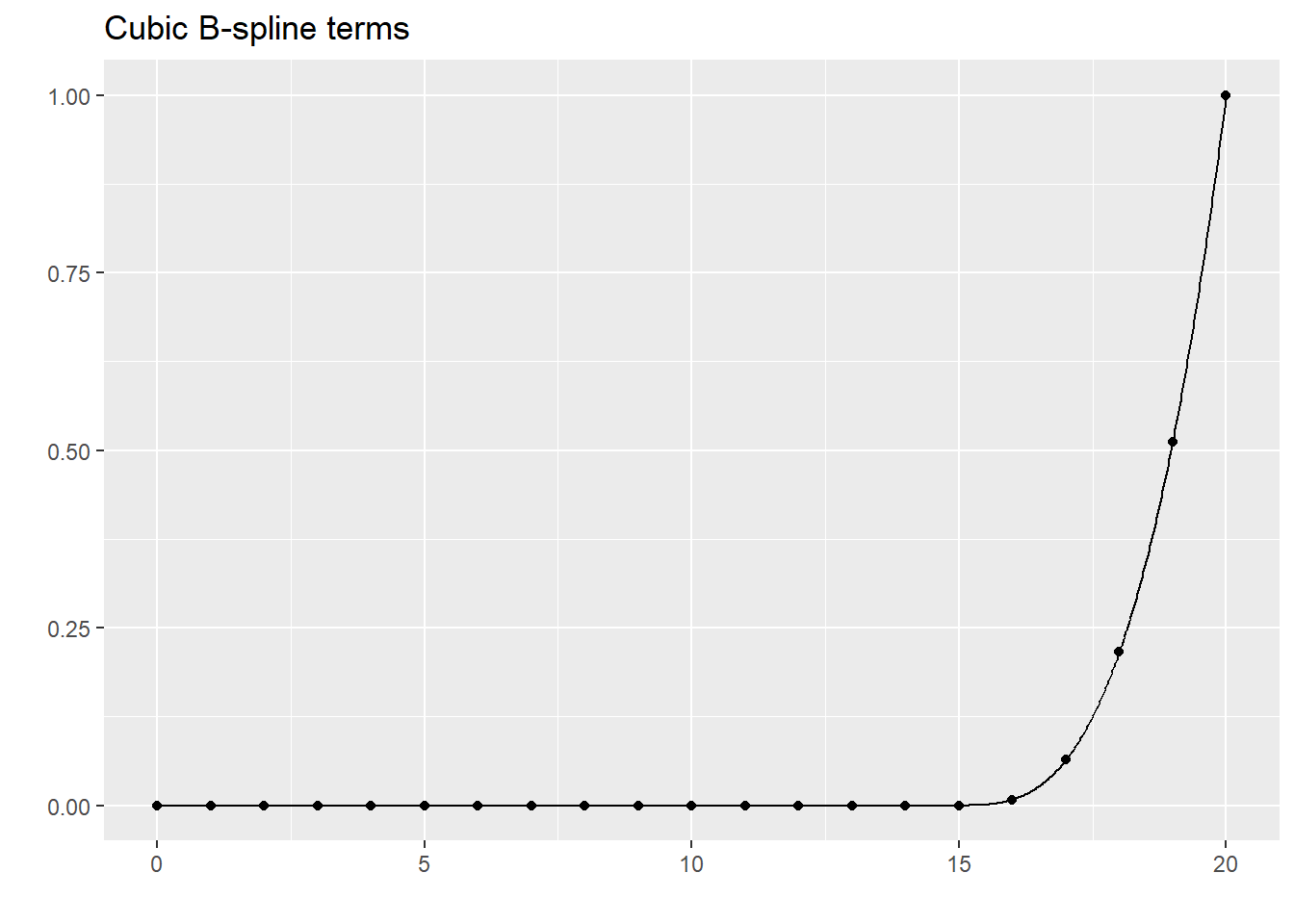B-splines have less issues with multicollinearity. round(cor(cubic_spline), 1) ## 1 2 3 4 5 6 7 ## 1 1.0 0.4 -0.2 -0.4 -0.3 -0.2 -0.1 ## 2 0.4 1.0 0.4 -0.5 -0.5 -0.4 -0.2 ## 3 -0.2 0.4 1.0 0.2 -0.6 -0.5 -0.3 ## 4 -0.4 -0.5 0.2 1.0 0.2 -0.5 -0.4 ## 5 -0.3 -0.5 -0.6 0.2 1.0 0.4 -0.2 ## 6 -0.2 -0.4 -0.5 -0.5 0.4 1.0 0.4 ## 7 -0.1 -0.2 -0.3 -0.4 -0.2 0.4 1.0 Although there is some correlation, this not nearly as bad as the piecewise approach. lm(xm$y~cubic_spline) %>%
augment(newdata=xm) %>%
select(c2, .fitted) %>%
display_curve("Cubic spline fit to the data") %>%
display_dots(simulated_example)
## Warning in predict.lm(x, newdata = newdata, na.action = na.pass, ...):
## prediction from a rank-deficient fit may be misleading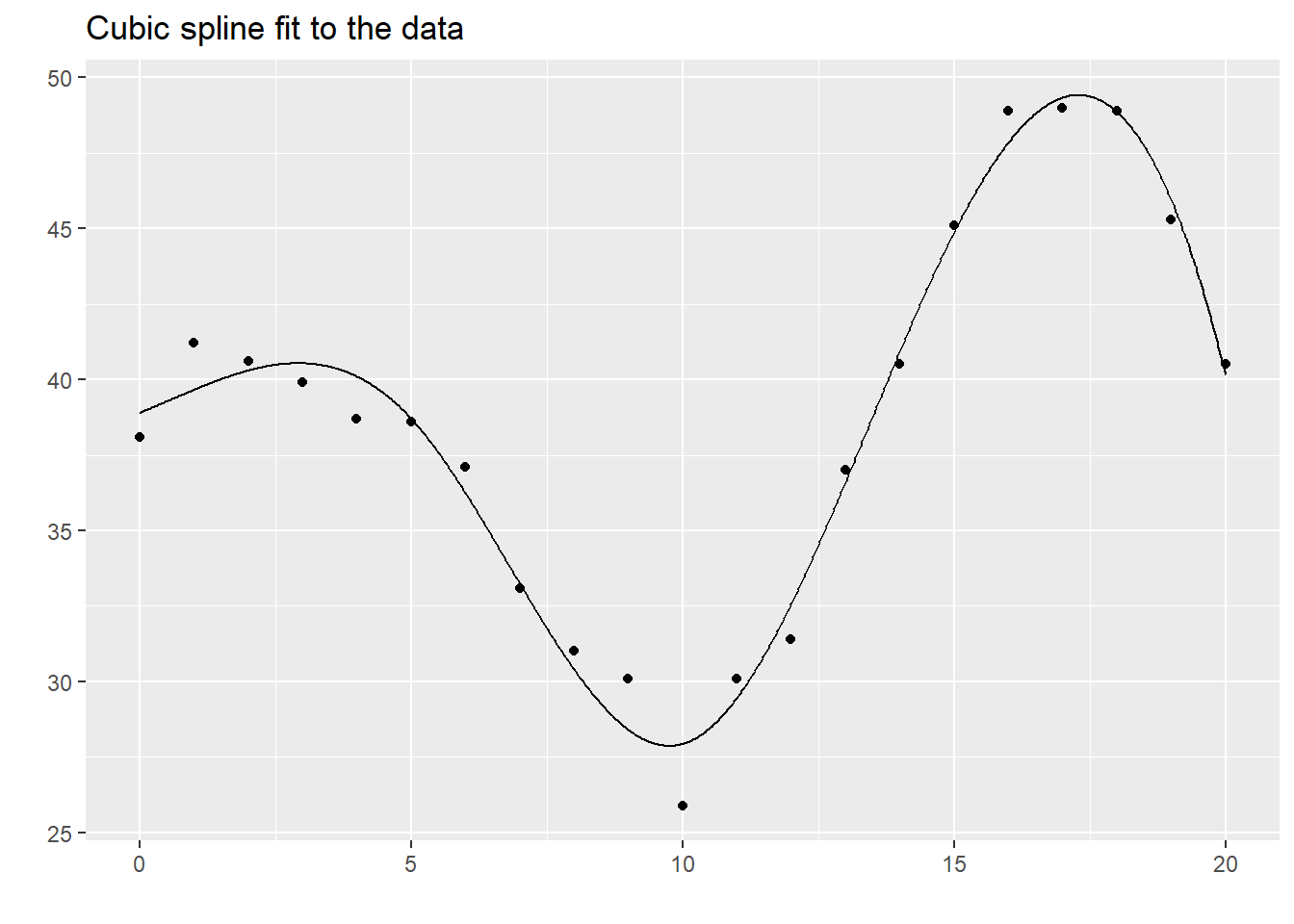Although most applications use cubic B-splines, they are available in other forms. Here is the linear spline.

linear_spline <- bs(xm$c2, knots=c(5, 10, 15), degree=1, intercept=TRUE) The terms in the linear B-splines are mostly piecewise linear functions. Notice how they are zero outside certain ranges, with only a small amount of overlap between adjacent terms. for (j in 1:dim(linear_spline)) { dat <- cbind(xm$c2, linear_spline[ , j])
display_curve(dat, "Linear B-spline terms") %>%
display_dots(dat[1:21, ]) %>%
plot
}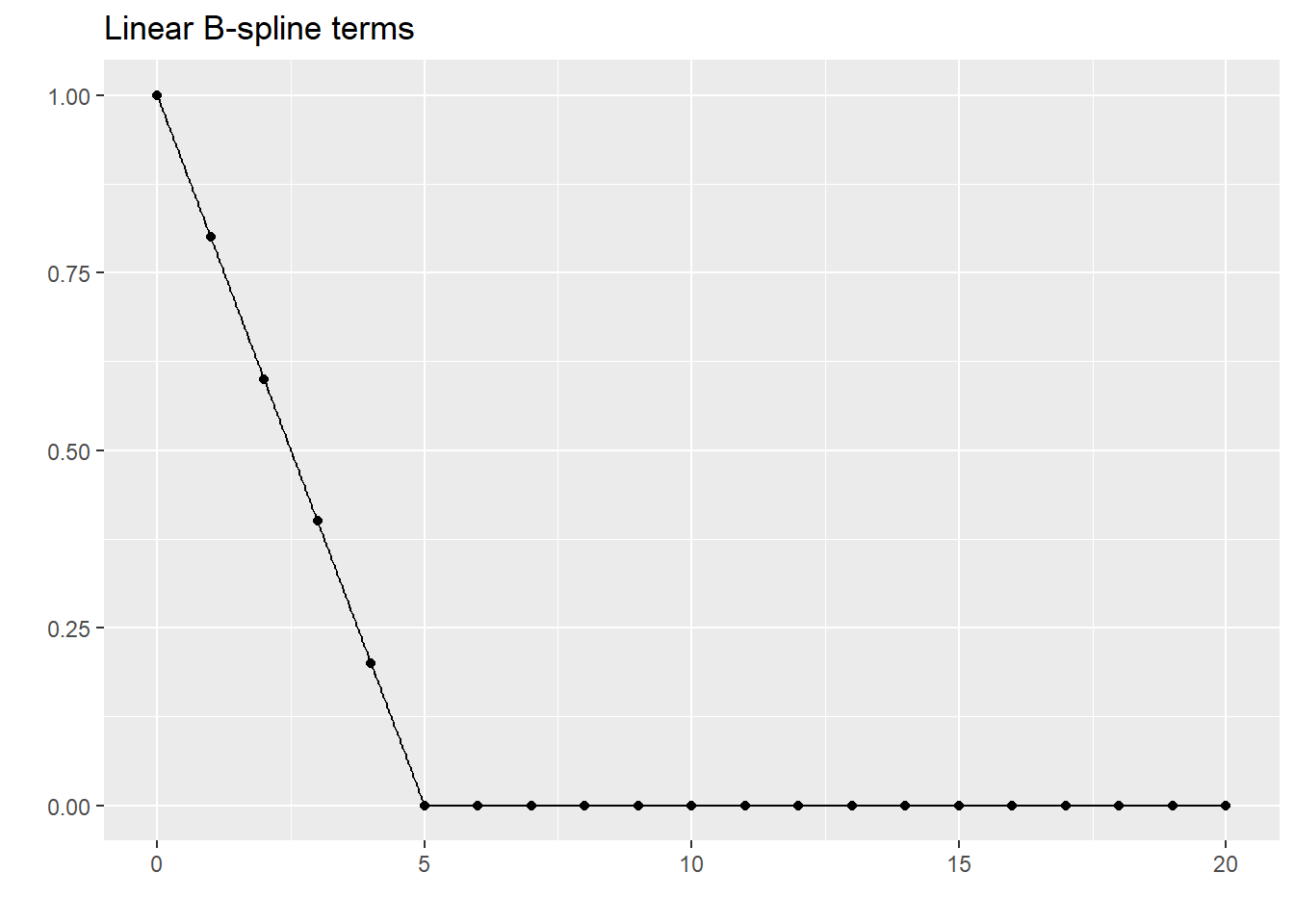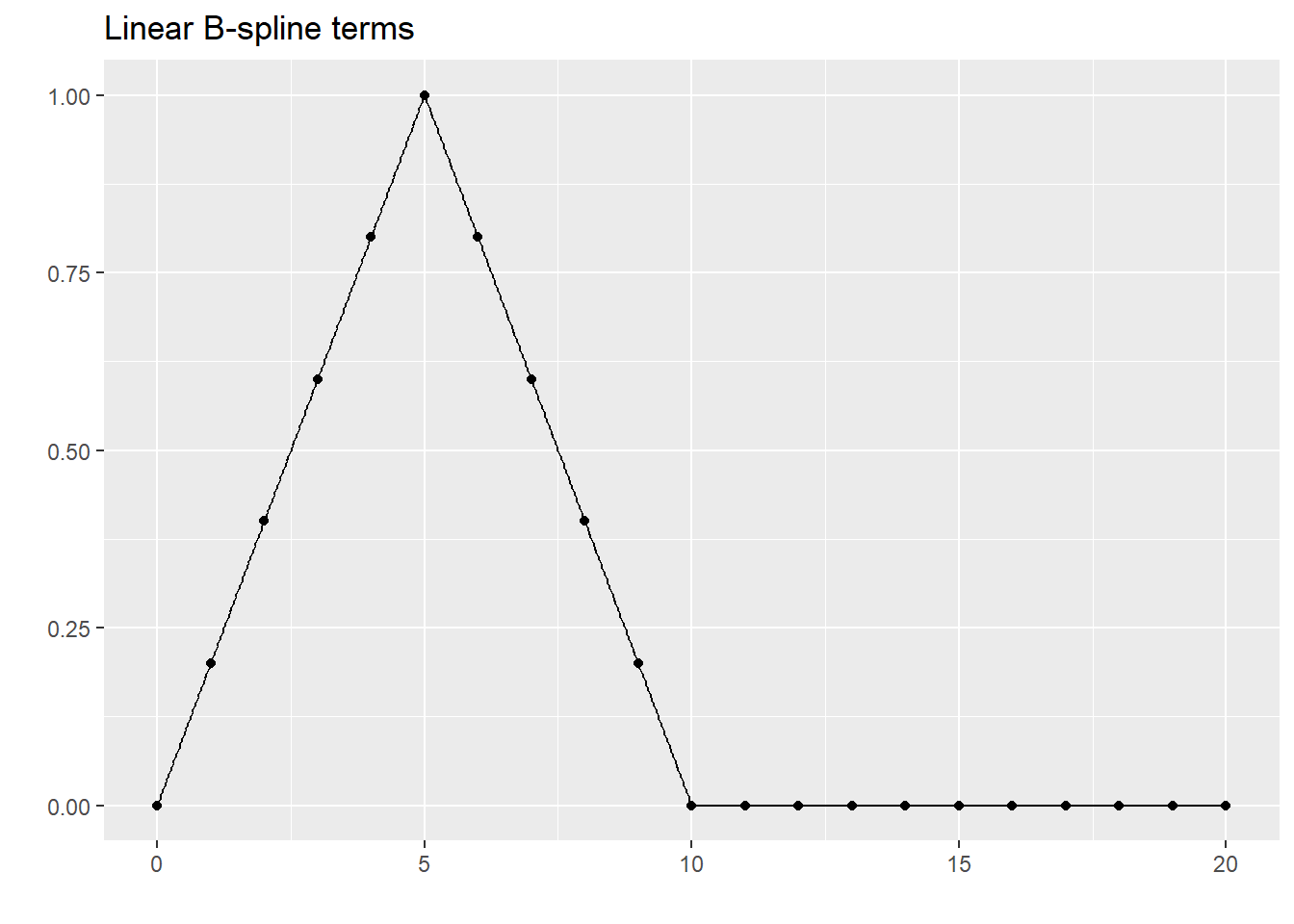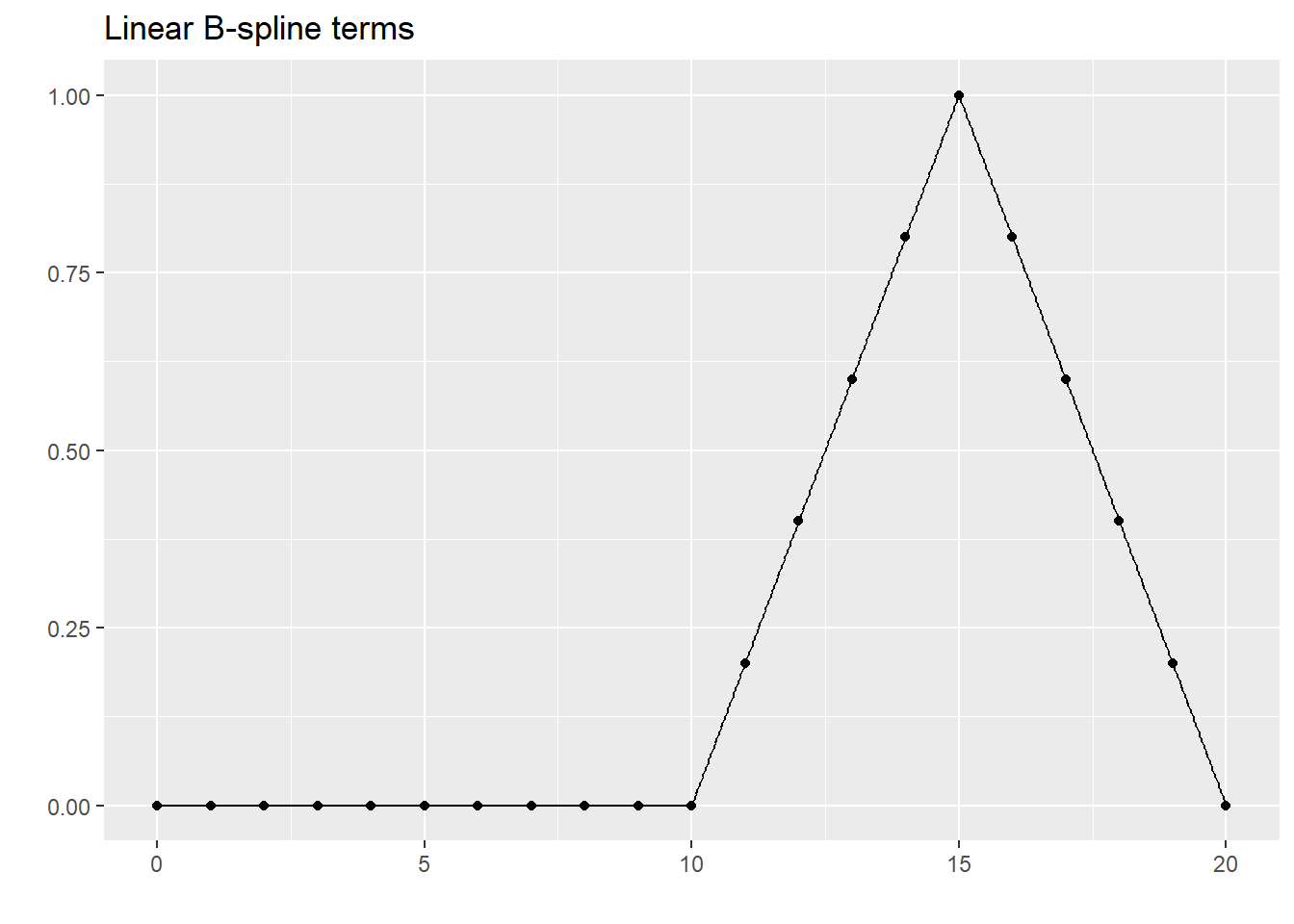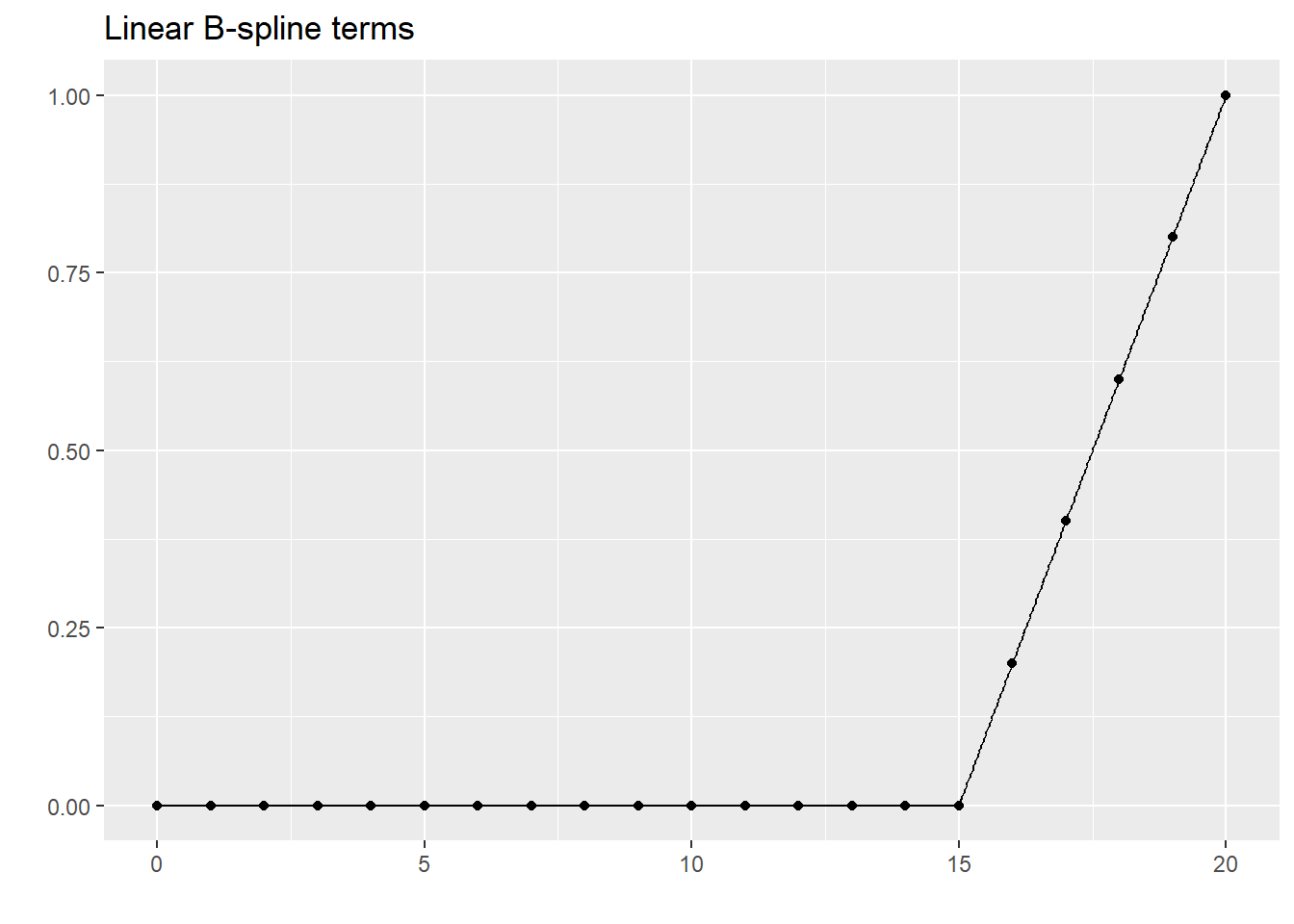This is a linear spline fit to the data you have been using.

lm(xm$y~linear_spline) %>% augment(newdata=xm) %>% select(c2, .fitted) %>% display_curve("Linear spline fit to the data") %>% display_dots(simulated_example) ## Warning in predict.lm(x, newdata = newdata, na.action = na.pass, ...): ## prediction from a rank-deficient fit may be misleadingA linear spline has continuity, but the nature of linear function makes it impossible to get smoothness. You can also compute quadratic splines. quadratic_spline <- bs(xm$c2, knots=c(5, 10, 15), degree=2, intercept=TRUE)
kable(quadratic_spline[1:21, ])
1 2 3 4 5 6
1.00 0.00 0.00 0.00 0.00 0.00
0.64 0.34 0.02 0.00 0.00 0.00
0.36 0.56 0.08 0.00 0.00 0.00
0.16 0.66 0.18 0.00 0.00 0.00
0.04 0.64 0.32 0.00 0.00 0.00
0.00 0.50 0.50 0.00 0.00 0.00
0.00 0.32 0.66 0.02 0.00 0.00
0.00 0.18 0.74 0.08 0.00 0.00
0.00 0.08 0.74 0.18 0.00 0.00
0.00 0.02 0.66 0.32 0.00 0.00
0.00 0.00 0.50 0.50 0.00 0.00
0.00 0.00 0.32 0.66 0.02 0.00
0.00 0.00 0.18 0.74 0.08 0.00
0.00 0.00 0.08 0.74 0.18 0.00
0.00 0.00 0.02 0.66 0.32 0.00
0.00 0.00 0.00 0.50 0.50 0.00
0.00 0.00 0.00 0.32 0.64 0.04
0.00 0.00 0.00 0.18 0.66 0.16
0.00 0.00 0.00 0.08 0.56 0.36
0.00 0.00 0.00 0.02 0.34 0.64
0.00 0.00 0.00 0.00 0.00 1.00

Notice how the quadratic spline terms transition smoothly to zero outside certain intervals.

for (j in 1:dim(quadratic_spline)) {
dat <- cbind(xm$c2, quadratic_spline[ , j]) display_curve(dat, "Quadratic B-spline terms") %>% display_dots(dat[1:21, ]) %>% plot }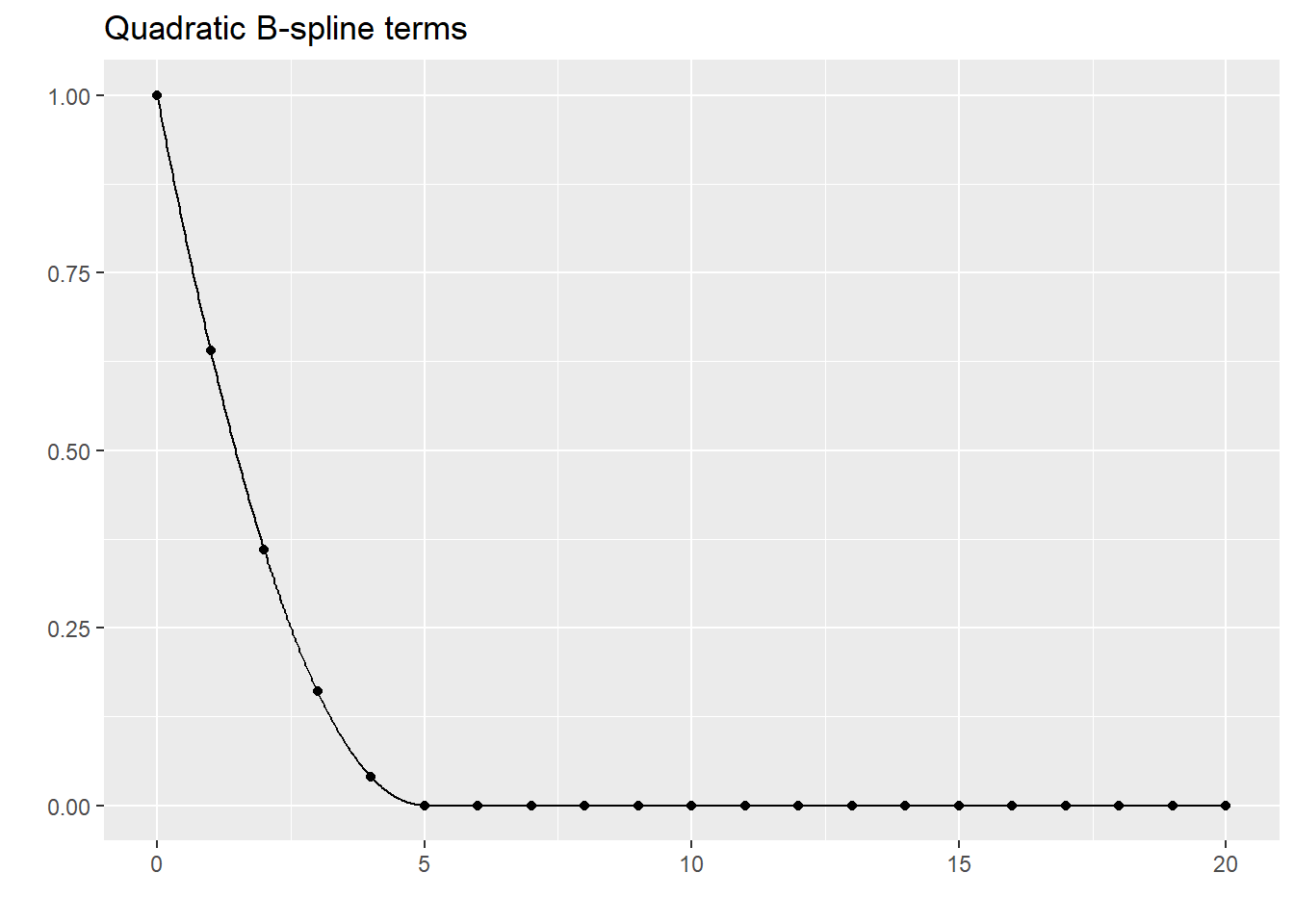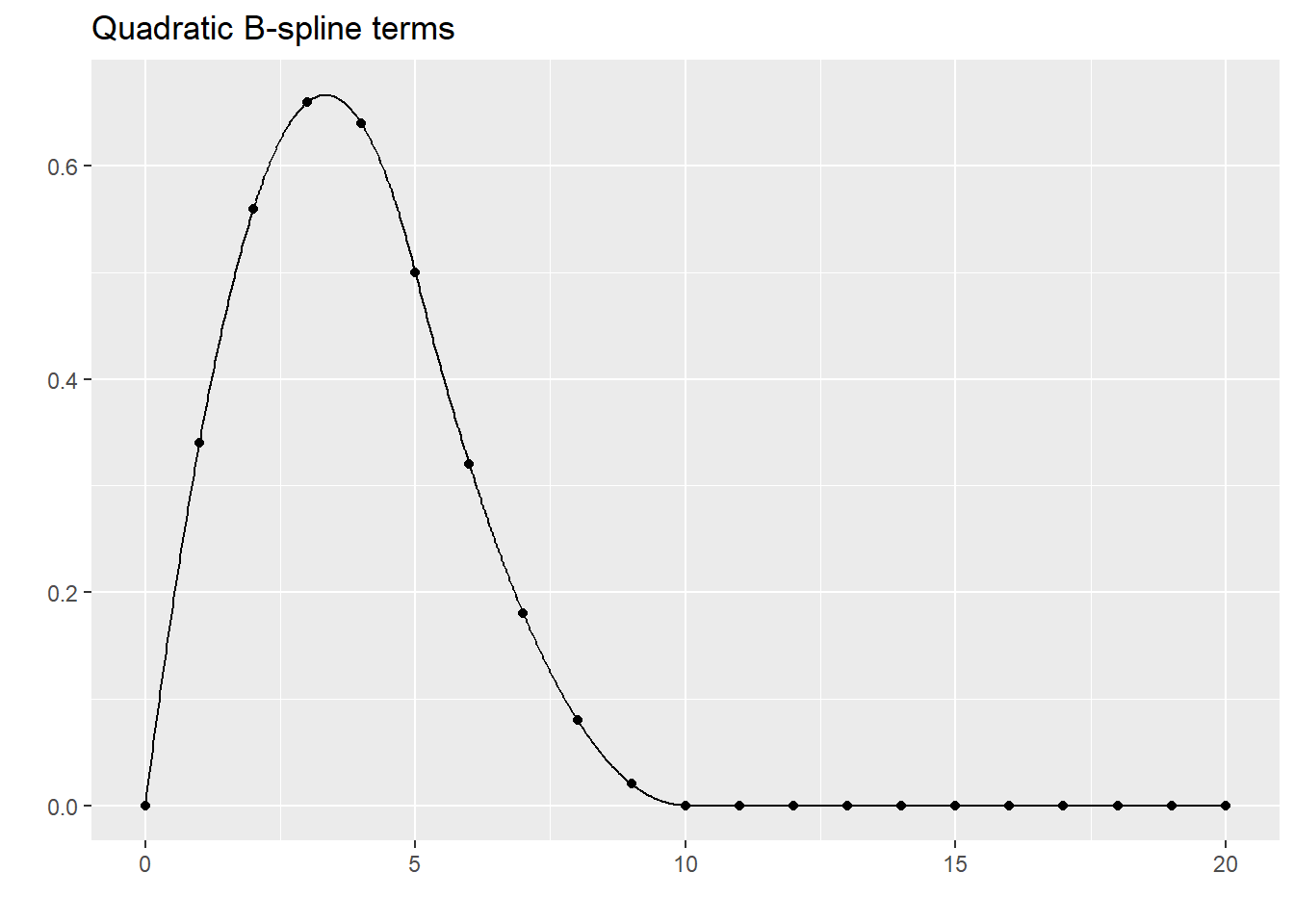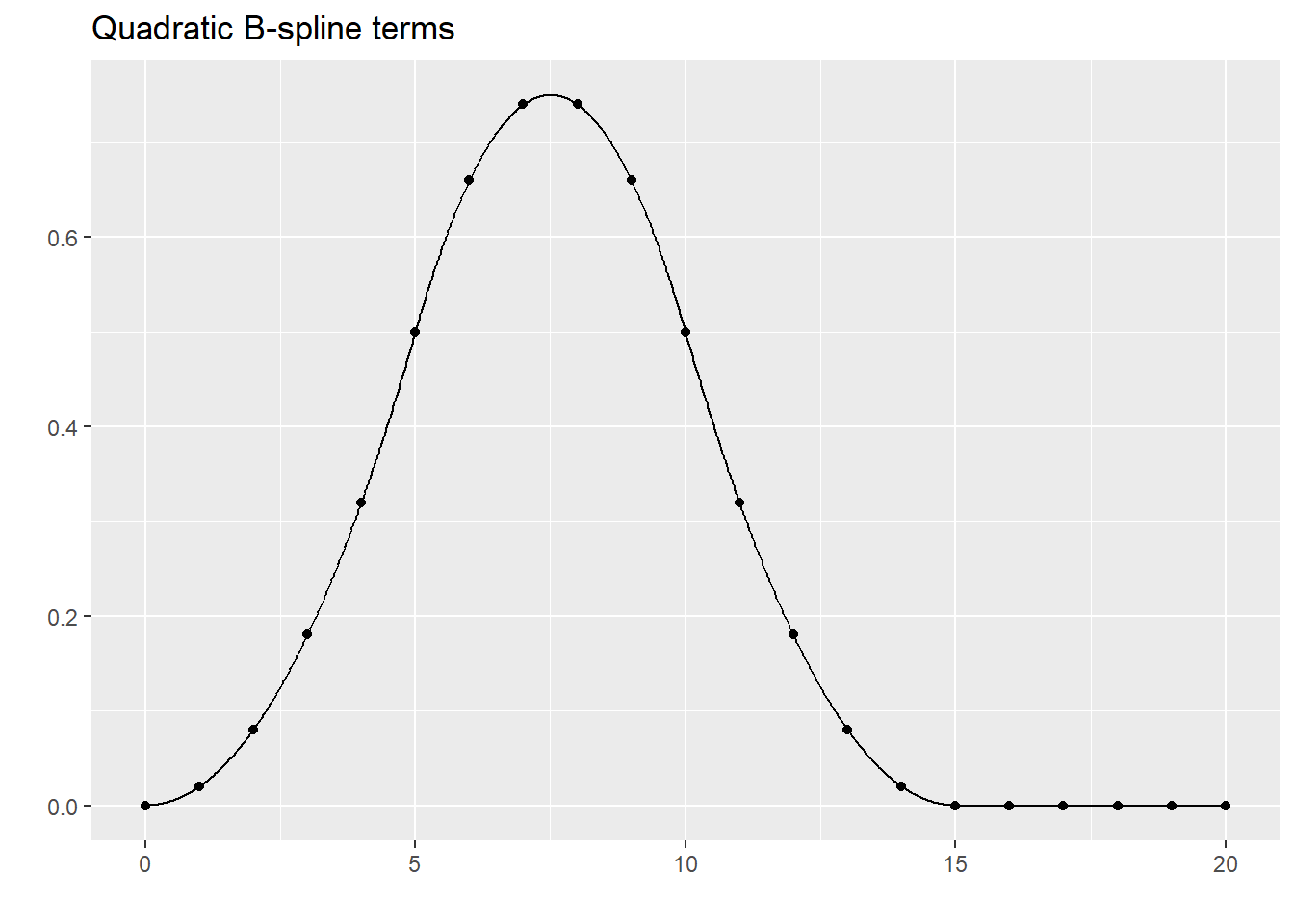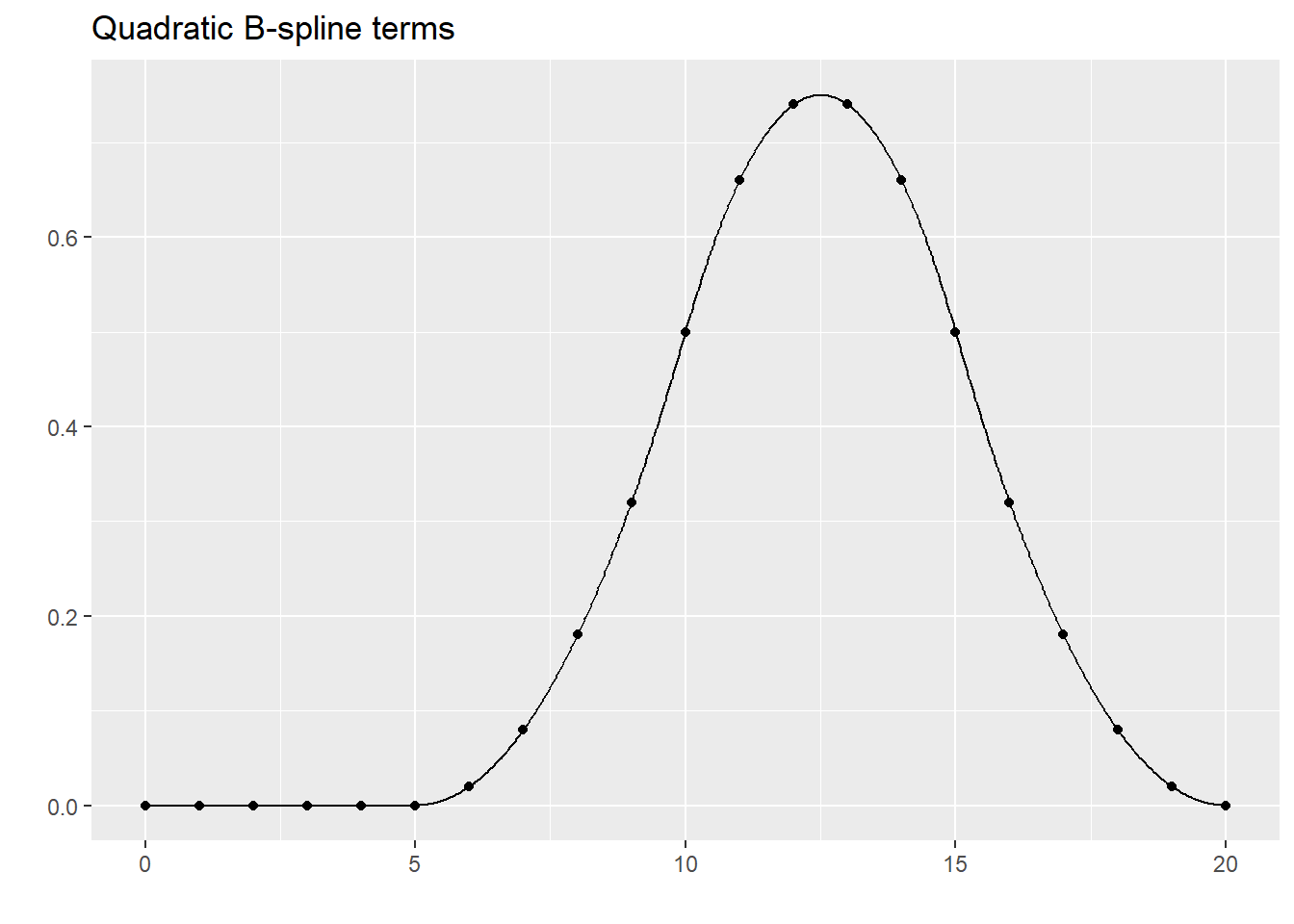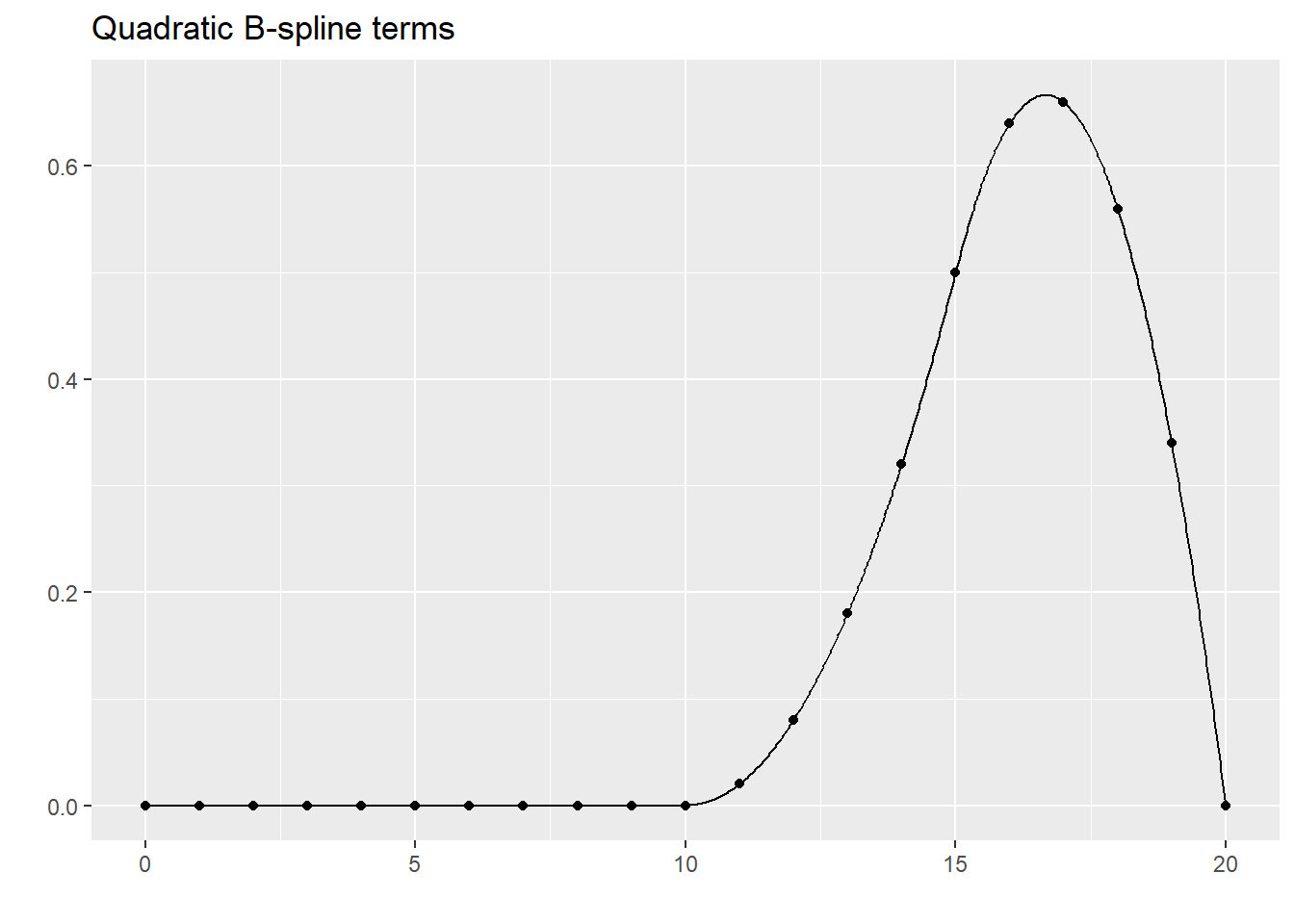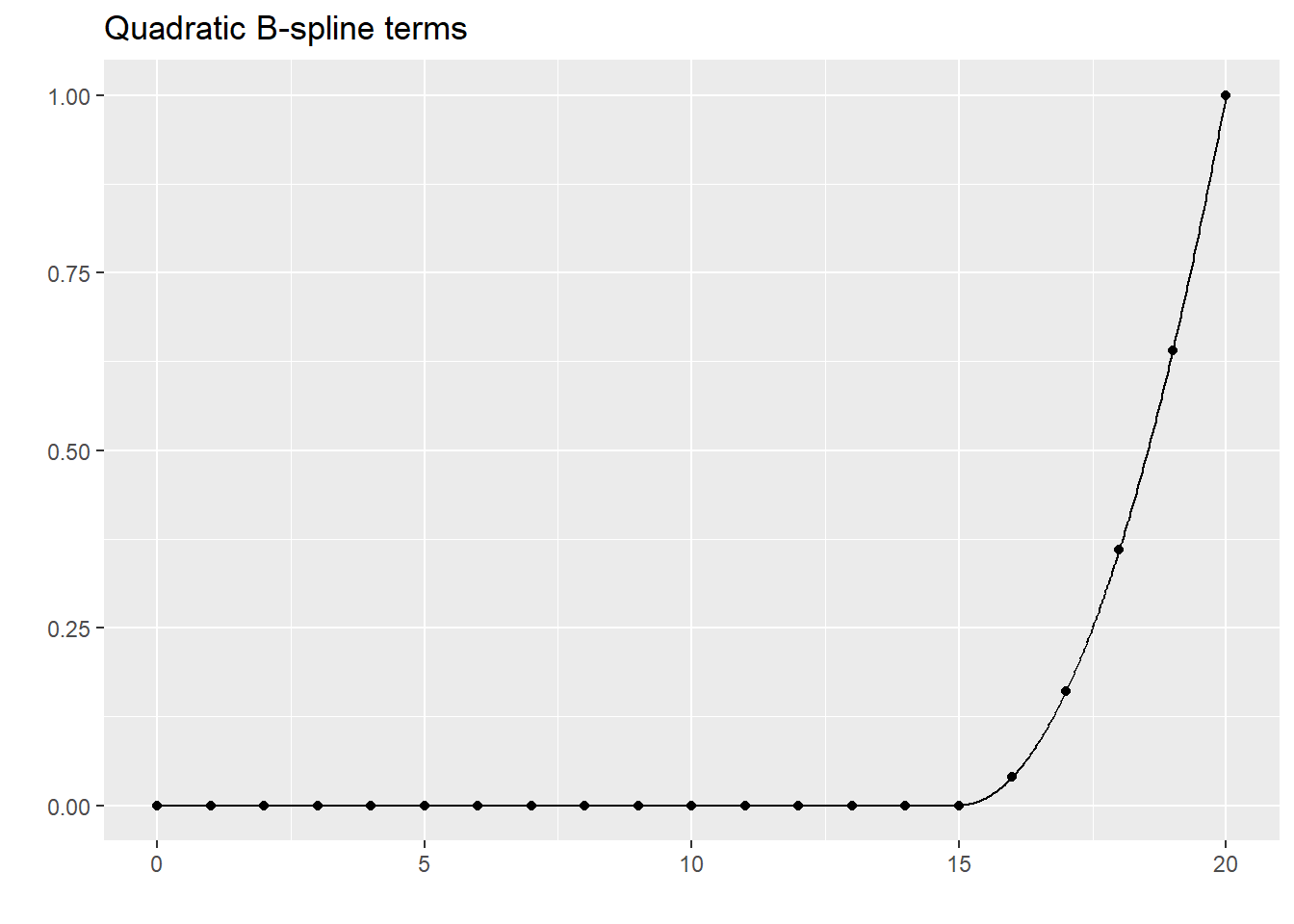This is the fit of the quadratic spline. It can only produce smoothness up to a continuous first derivative, but the difference between this and smoothness with continuous first and second derivatives is subtle. lm(xm$y~quadratic_spline) %>%
augment(newdata=xm) %>%
select(c2, .fitted) %>%
display_curve("Quadratic spline fit to the data") %>%
display_dots(simulated_example)
## Warning in predict.lm(x, newdata = newdata, na.action = na.pass, ...):
## prediction from a rank-deficient fit may be misleading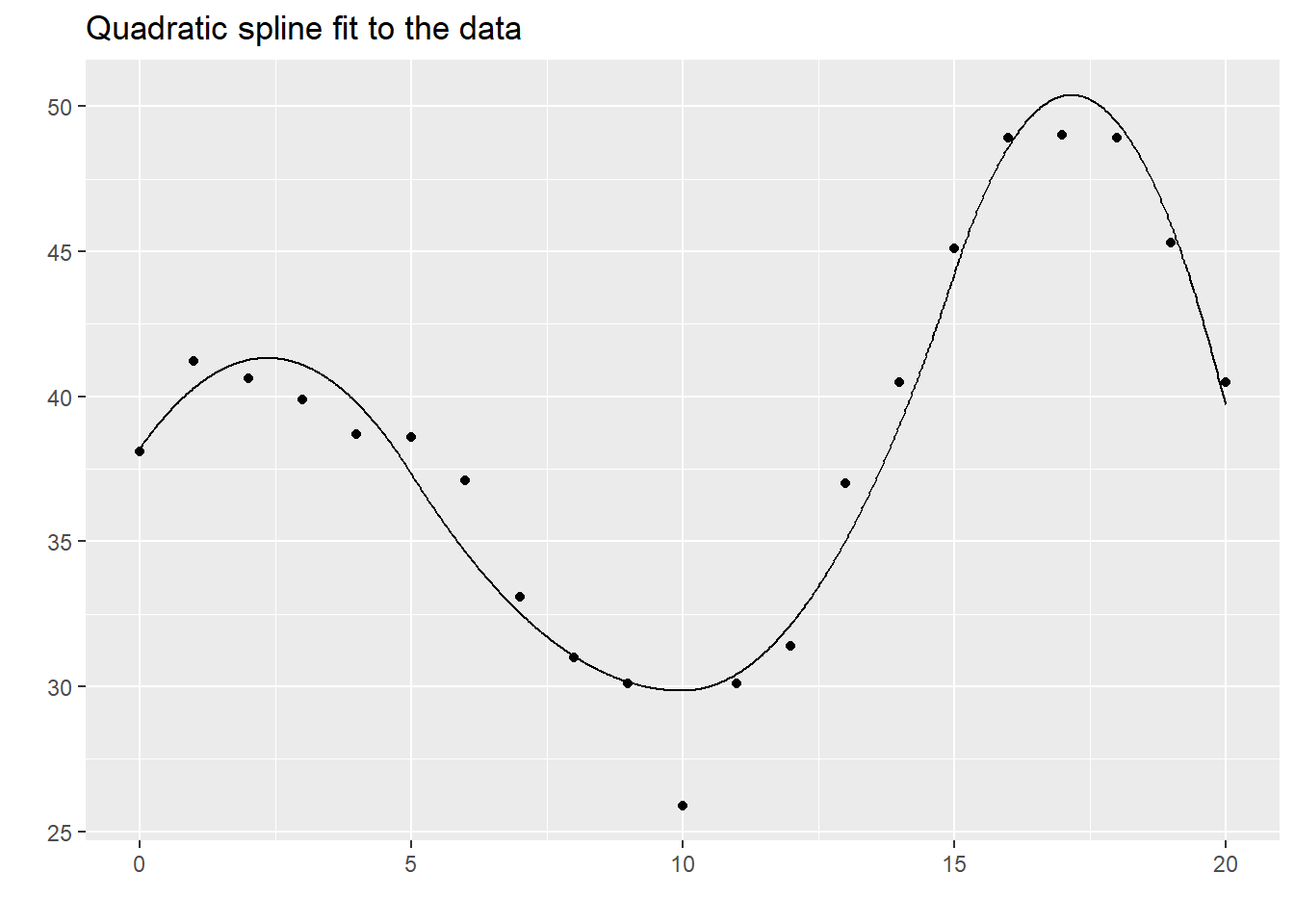The quadratic spline seems to do fairly well here and there is often little difference between it and the cubic spline. Nevertheless, cubic splines are far more common.

You could also look at quartic splines (using fourth order polynomials) or even higher. Sometimes these are used when you need to examine not just the function itself, but its derivatives. These applications, however, are rare.

### Natural splines

A variant on B splines are natural splines (also called restricted cubic splines). These splines place an additional restriction to the left of the first knot and to the right of the last knot. The spline is constrained to be linear at both extremes. This makes practical sense, as there is less data at the extremes, making estimation of a complex cubic function here worrisome. This also makes extrapolation outside of the range of data less problematic. Cubic polynomials have the potential of extreme shifts and if these occur outside the range of the data, they could lead to some awful extrapolations.

You should always be very careful, of course, as any effort to extrapolate beyond the range of data is dangerous. Nevertheless, restricting the extrapolation to a linear function is probably safer than letting the cubic polynomial wiggle around.

natural_spline <- ns(xm$c2, knots=c(5, 10, 15), intercept=TRUE) kable(natural_spline[1:21, ]) 1 2 3 4 5 -0.2672612 0.0000000 -0.2142857 0.6428571 -0.4285714 -0.0260174 0.0013333 -0.1790790 0.5372370 -0.3581580 0.1978331 0.0106667 -0.1449894 0.4349683 -0.2899789 0.3868969 0.0360000 -0.1131342 0.3394025 -0.2262684 0.5237807 0.0853333 -0.0846304 0.2538911 -0.1692607 0.5910913 0.1666667 -0.0605951 0.1817853 -0.1211902 0.5785043 0.2826667 -0.0405849 0.1257546 -0.0838364 0.5039715 0.4146667 -0.0179132 0.0857397 -0.0571598 0.3925141 0.5386667 0.0156670 0.0609990 -0.0406660 0.2691528 0.6306667 0.0684029 0.0507913 -0.0338608 0.1589087 0.6666667 0.1485417 0.0543749 -0.0362499 0.0813613 0.6306667 0.2591010 0.0706971 -0.0457981 0.0343243 0.5386667 0.3821802 0.0974593 -0.0543062 0.0101702 0.4146667 0.4946495 0.1320514 -0.0520343 0.0012713 0.2826667 0.5733788 0.1718636 -0.0292424 0.0000000 0.1666667 0.5952381 0.2142857 0.0238095 0.0000000 0.0853333 0.5436190 0.2571429 0.1139048 0.0000000 0.0360000 0.4280000 0.3000000 0.2360000 0.0000000 0.0106667 0.2643810 0.3428571 0.3820952 0.0000000 0.0013333 0.0687619 0.3857143 0.5441905 0.0000000 0.0000000 -0.1428571 0.4285714 0.7142857 It is a bit tricky to show the extrapolation of nte natural splines. This approach uses the predict function. extended_x <- seq(-5, 25, by=1/64) extended_spline <- predict(natural_spline, extended_x) for (j in 1:dim(extended_spline)) { dat <- cbind(extended_x, extended_spline[ , j]) spline_dots <- cbind(0:20, extended_spline[extended_x %in% 0:20, j]) display_curve(dat, "Natural spline terms") %>% display_dots(spline_dots) %>% plot }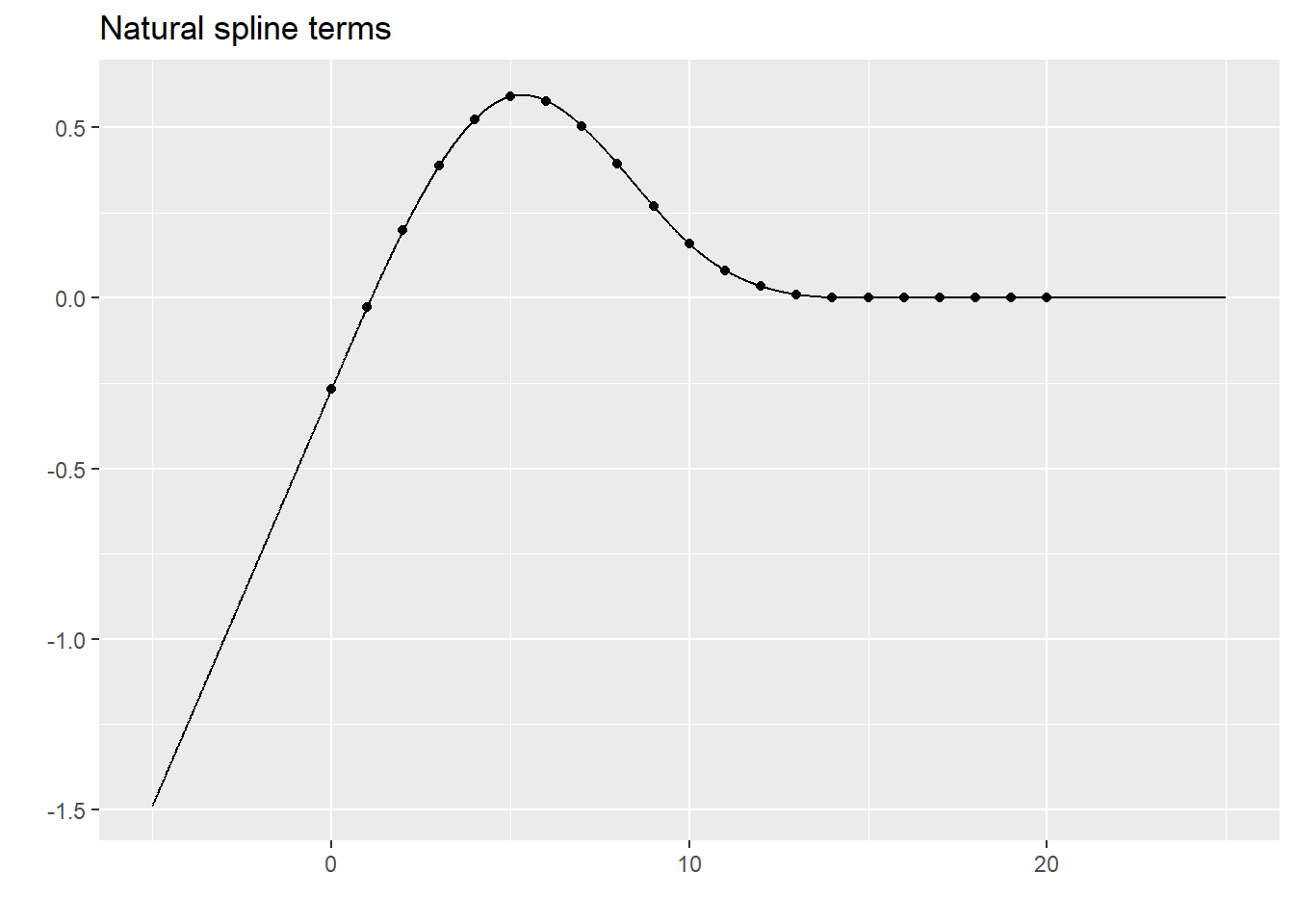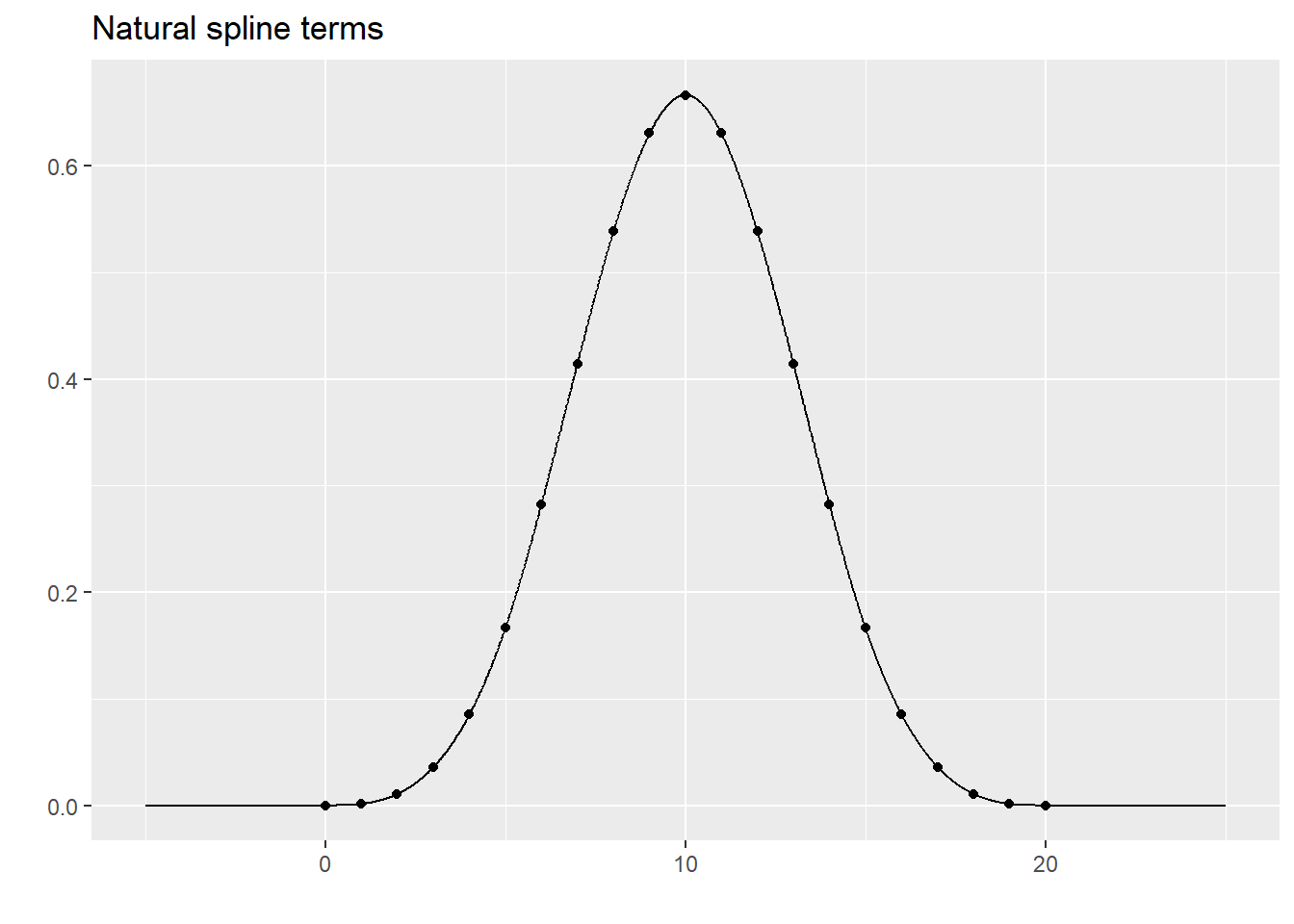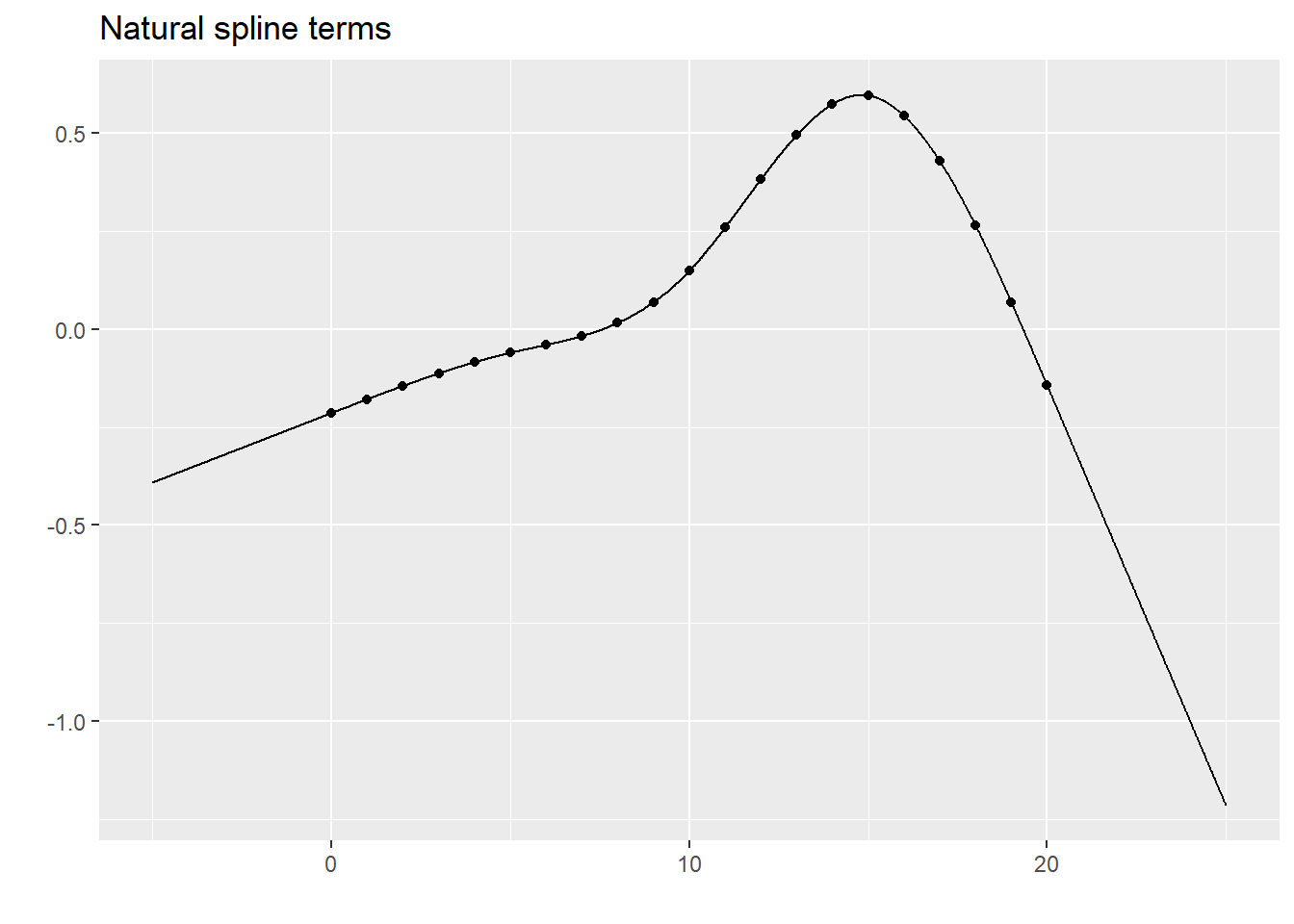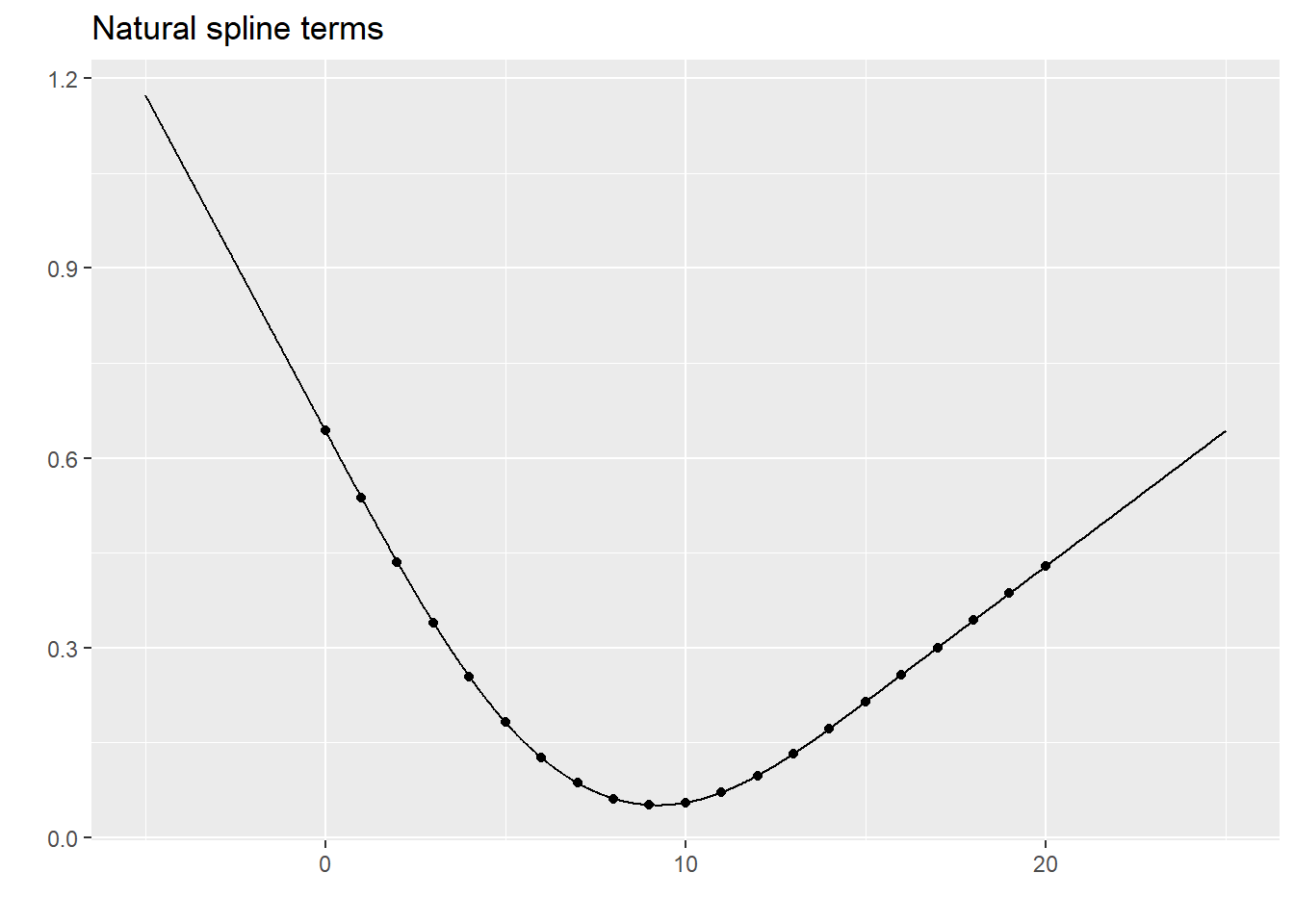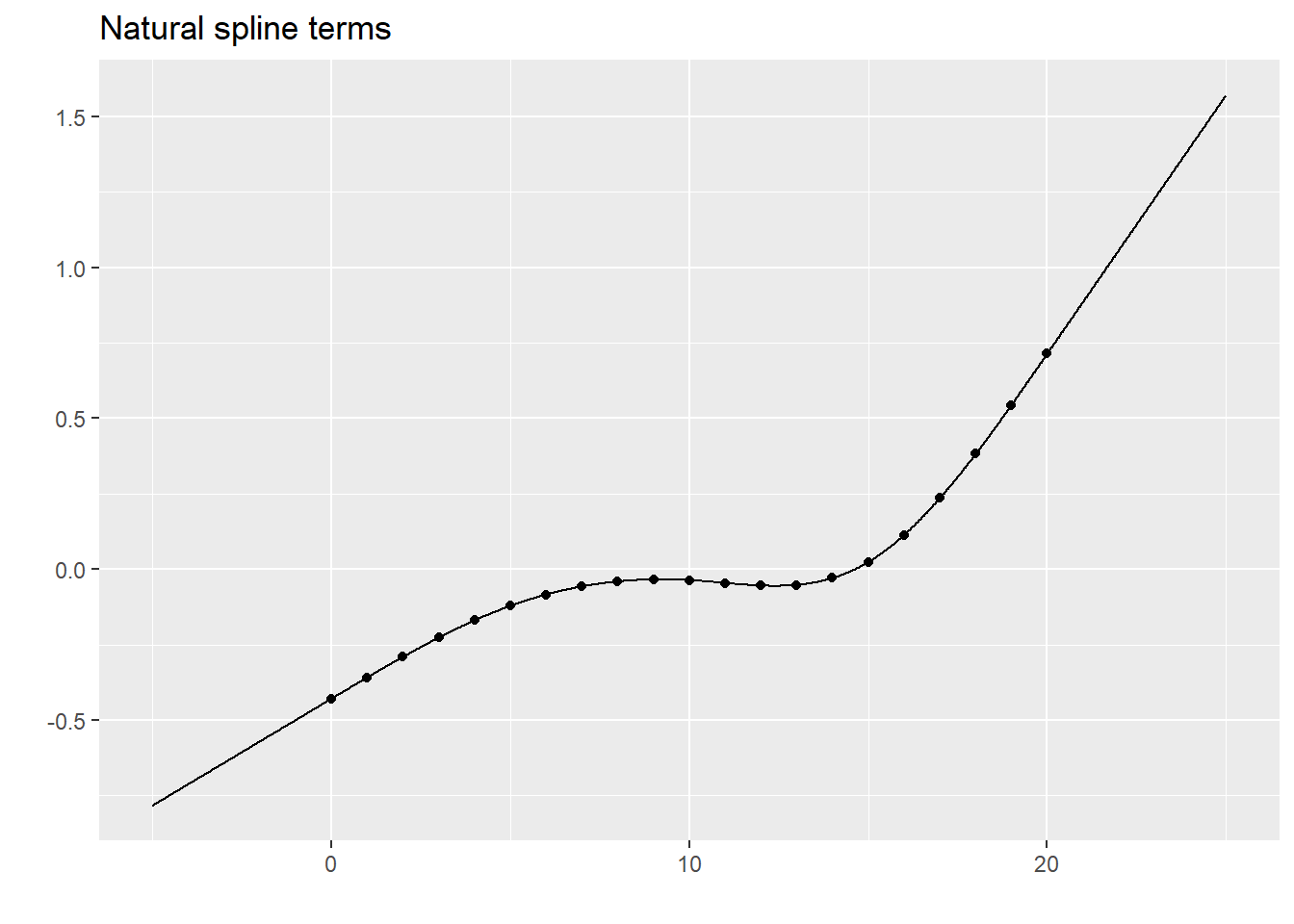Here is the natural spline fit to your simulated dataset. extended_y <- rep(NA, length(extended_x)) extended_y[extended_x %in% 0:20] <- simulated_example$y
lm(extended_y~extended_spline) %>%
augment(newdata=extended_spline) %>%
data.frame(c2=extended_x) %>%
select(c2, .fitted) %>%
display_curve("Natural spline fit to the data") %>%
display_dots(simulated_example)
## Warning in predict.lm(x, newdata = newdata, na.action = na.pass, ...):
## prediction from a rank-deficient fit may be misleading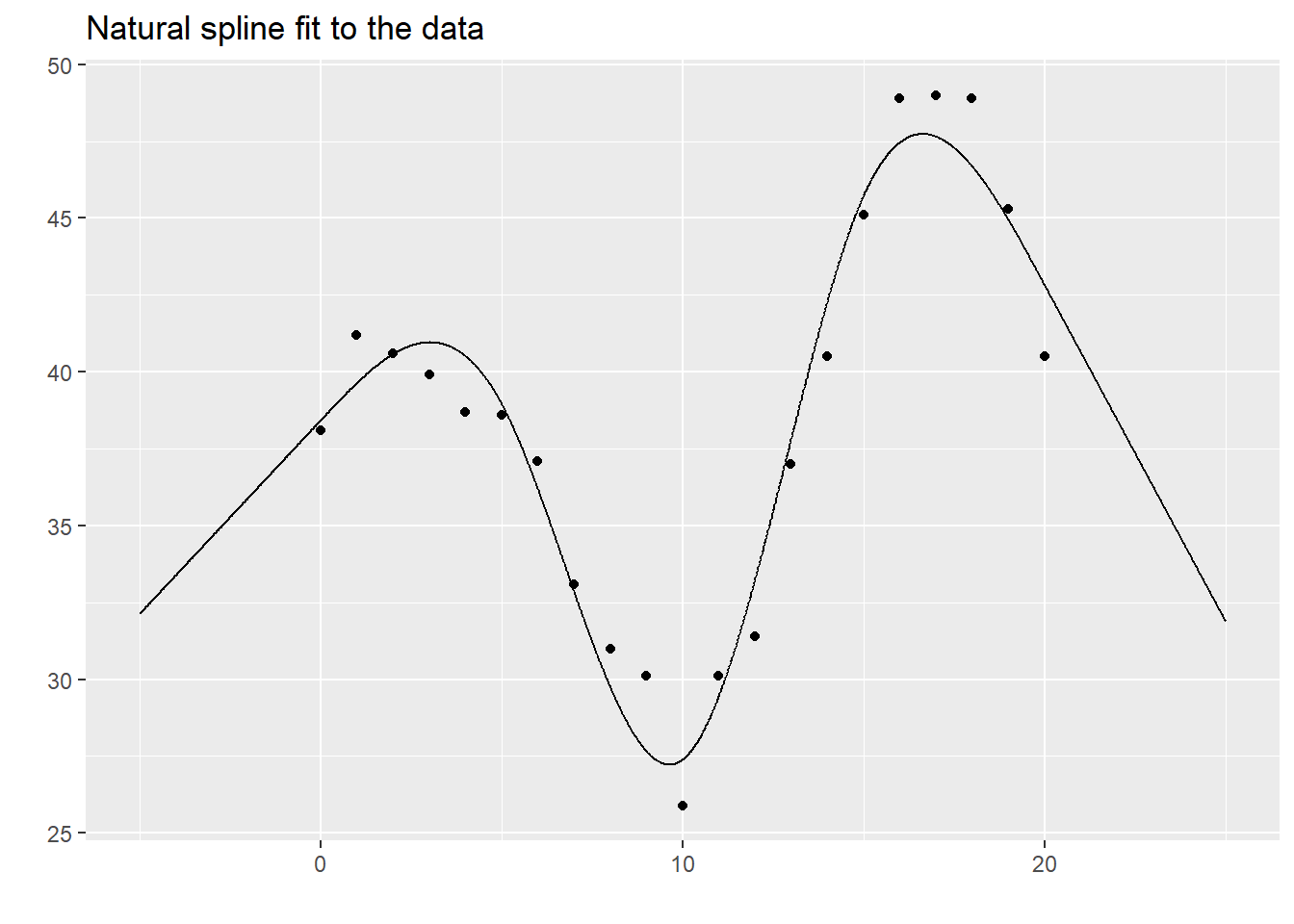You can probably see the linearity at the extremes of the data.

### How many knots?

A difficult question is how many knots to use. Too many knots and you might end up overfitting. Too few and you might end up with not enough flexibility to fit your data well.

#### Use AIC or BIC

The AIC (Akaike Information Criterion) and the BIC (Bayesian Information Criterion) are useful measures for comparing different statistical models. In linear regression both AIC and BIC look at how close the fitted curve is to the data, but adds a penalty for model complexity. This helps avoid the situation where an excessively complex model with only marginally better predictive power is selected over a simpler model that predicts almost as well.

NOte that a p-value will not work here except in some special cases where all of the knots but one coincide. The p-value fails because (with a few rare exceptions) one spline model is not nested inside another.

for (k in 3:7) {
knots <- 20*((1:k)-0.5)/k
lm(y~bs(c2, knots=knots), data=xm) -> rcs_fit
# lm(y~rcs(c2, k), data=xm) -> rcs_fit
ti <- paste0(k, " knots, AIC = ", round(AIC(rcs_fit), 1), ", BIC = ", round(BIC(rcs_fit), 1))
rcs_fit %>%
augment(newdata=xm) %>%
select(c2, .fitted) %>%
display_curve(ti) %>%
display_dots(simulated_example) -> cplot
plot(cplot + geom_vline(xintercept=knots, color="black", linetype="dotted"))
}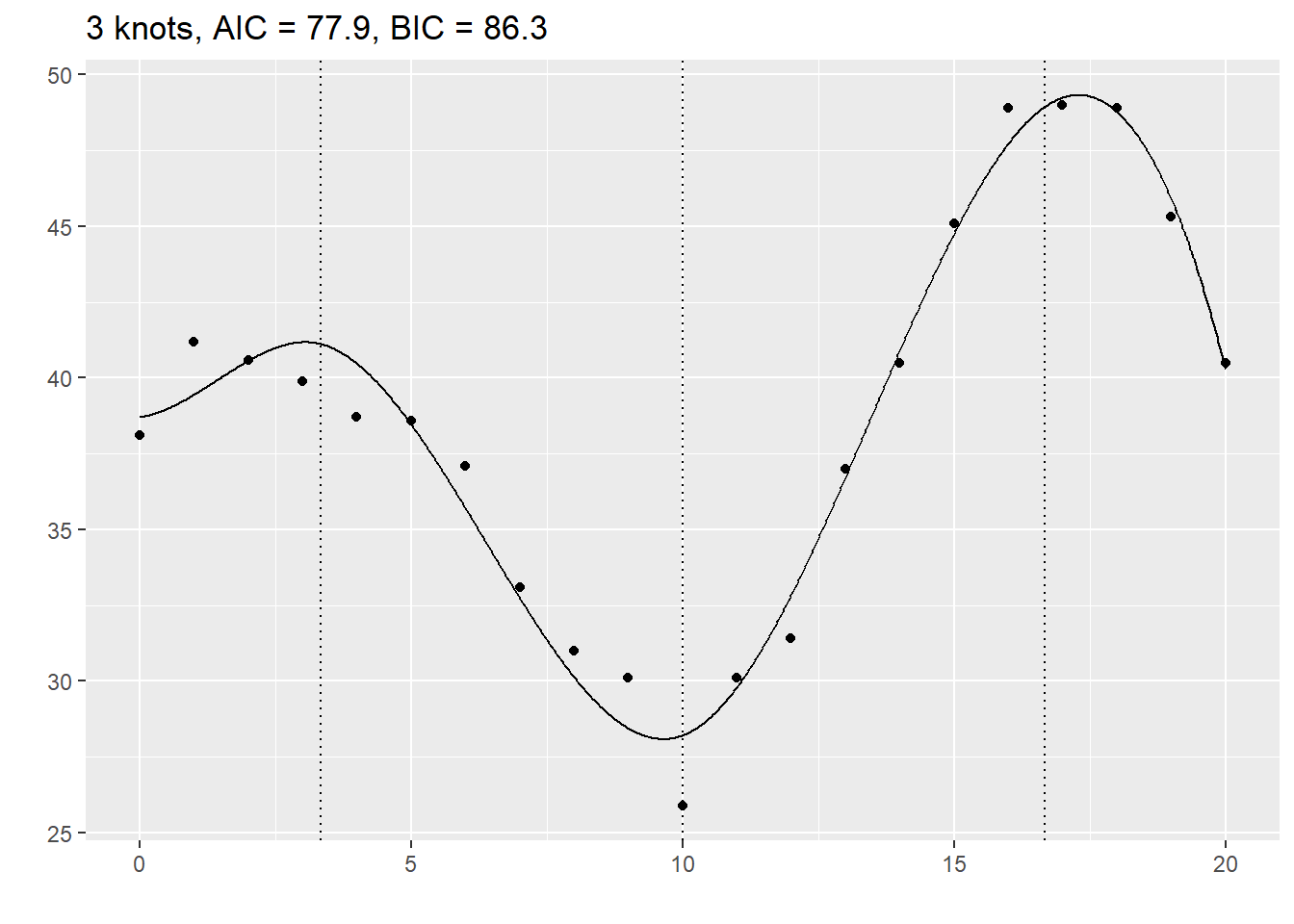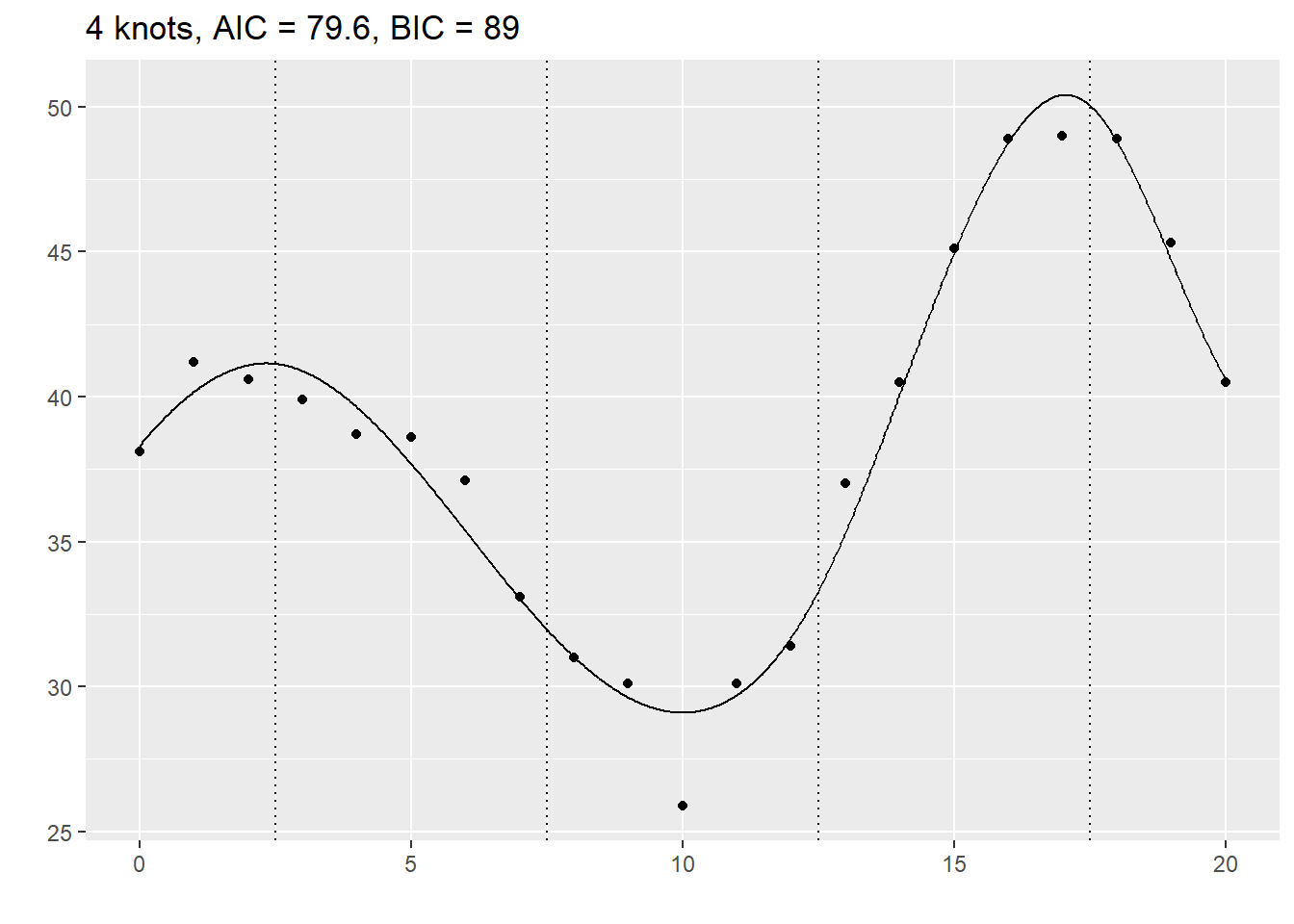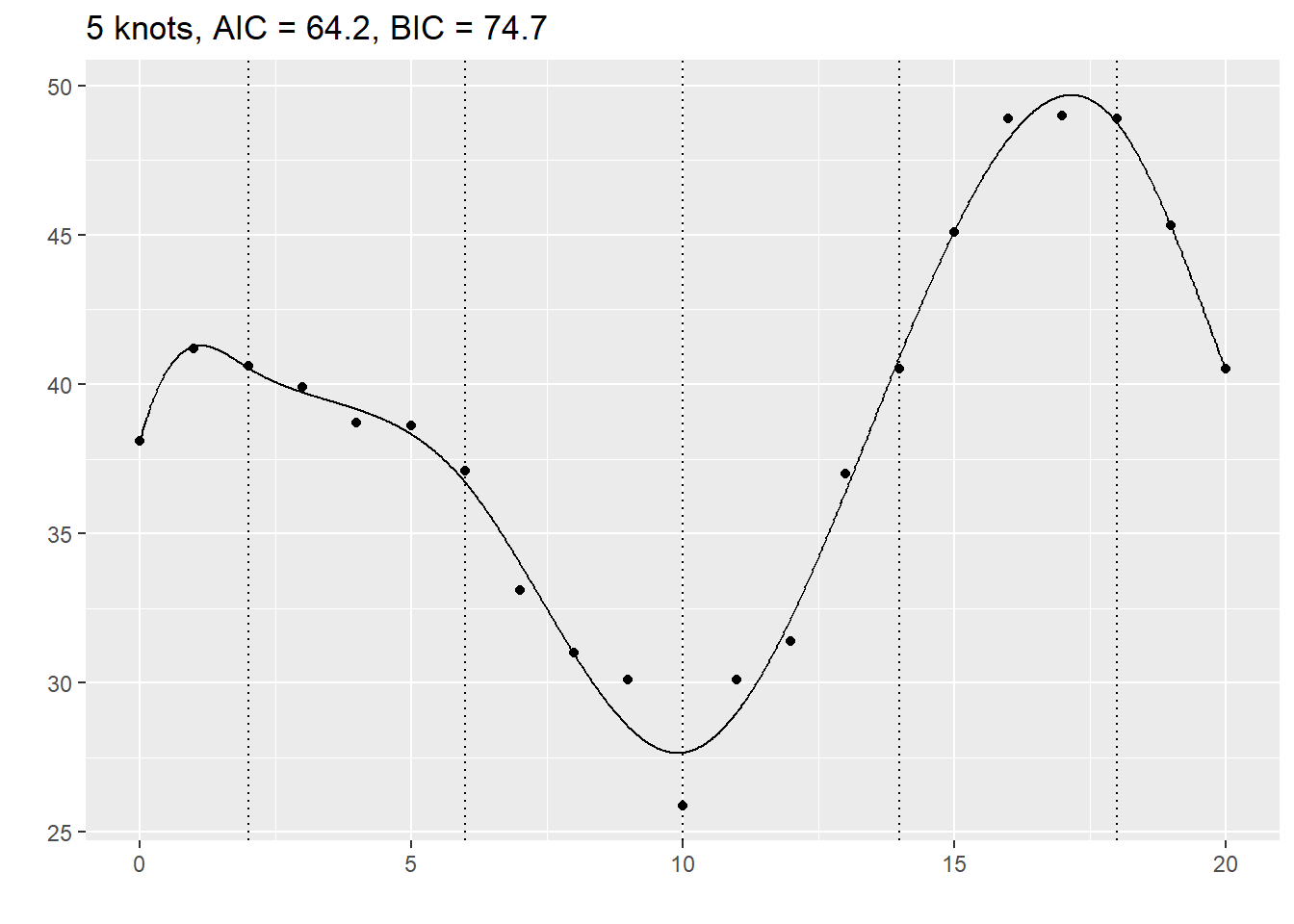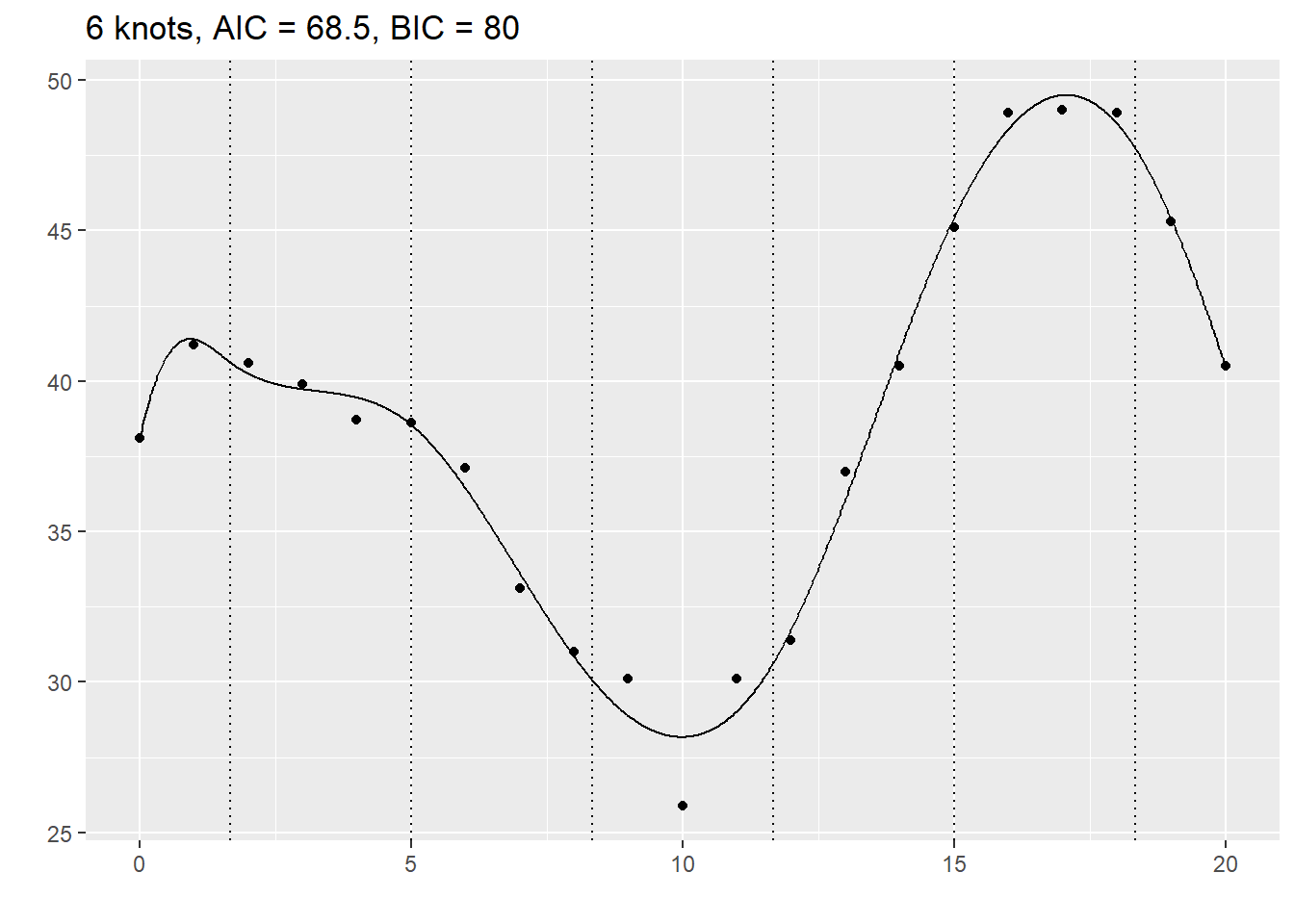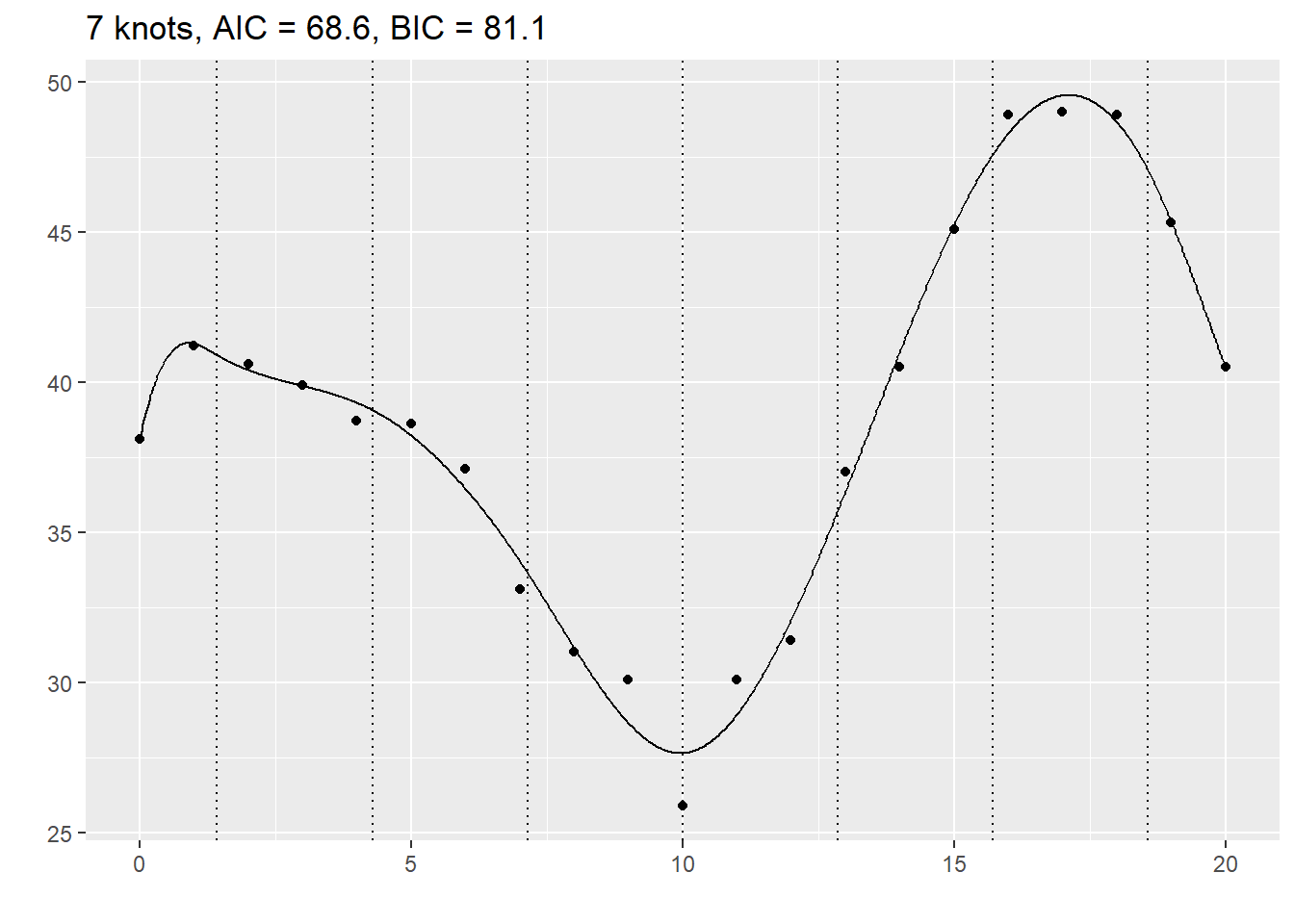#### Eyeball the data

Look at the number of bends in the data. If the data increases to a single maximum and then decreases after that, a simpler spline with 2 or 3 knots may be sufficient. This also applies if the data decreases to a single minimum and then increases after that. If there are more bends (e.g., increase to a maximum, decrease to a minimum, and then increase again), then a larger number of knots may be needed.

#### Frank Harrell’s suggestions

Use 4 knots if the total sample size is less than 100 and use 5 knots if it is more than 100.

#### Pick a number based on your a priori beliefs

You may have a feel for how much complexity is appropriate based on your years of experience as a data analyst and your scientific knowledge of the process at hand. After you work with enough splines, you do get an appreciation on how wiggly they can get. If you also have a rough idea of how the nonlinear relationship is going to be, perhaps based on seeing other similar problems in the area, you can match the degrees of freedom of the spline to your expectation, prior to looking at the data.

#### Pick a number after looking at some preliminary graphs

This is a bit controversial. Selecting a statistical model post hoc (after viewing the data) leaves you open to a charge of data dredging or going on a fishing expedition. To be fair, it is not as bad as some approaches (such as running ten tests and then choosing the one with the smallest p-value).

### Where to place the knots?

#### Use inside knowledge

Sometimes you have knowledge of the specific application that will help you to figure out where to put your knots.

I am not an expert on cars, but I have been told that many newer cars with automatic transmission change how the transmission behaves around 40 miles per hour. This transition helps with highway mileage. So if you are fitting a spline curve to data where how the transmission behaves, make sure that you place one or two of your knots near 40 miles per hour.

I am also not an expert on kidneys, but I have been told that the Glomerural Filtration Rate is not too critical if the value is above 90, but becomes very serious when it is less than 30. So a model looking at health effects using GFR should probably have knots around 30 and 90.

Similarly, CD4 cell counts above 500 are a good sign, but things turn rapidly worse if they dip below 200.

#### Frank Harrell’s suggestion

Frank Harrell suggests that you place the knots not evenly across the range of X but at equally spaced quantiles of the X distribution. This makes sense when the distribution of the X values is not uniform. If, for example, X is skewed to the right (has a tendency to produce most of the data on the left with a few scattered outliers on the right), the knots will tend to favor the data-rich left side of the distribution. He also suggests placing the leftmost and rightmost knots near, but not at the extremes of the X values, such as at the 10th or 90th percentiles or at the fifth smallest and the fifth largest values in the data. The actual percentiles are a bit tricky to explain.

“For 3 knots, the outer quantiles used are 0.10 and 0.90. For 4-6 knots, the outer quantiles used are 0.05 and 0.95. For more than 6 knots, the outer quantiles are 0.025 and 0.975. The knots are equally spaced between these on the quantile scale. For fewer than 100 non-missing values of x, the outer knots are the 5th smallest and the 5th largest x.” as quoted here,

If you pick this apart, you can deduce that 4 knots for a large dataset would be placed at the 5th, 35th, 65th and 95th percentiles.

#### It doesn’t matter

Most references I have looked at state that it is the number of knots rather than the placement of the knots that is critical.

Here are B-spline models with knots sliding from 3, 8, 13 to 7, 12, 17.

for (k in 3:7) {
knots <- k + c(0, 5, 10)
lm(y~bs(c2, knots=knots), data=xm) -> rcs_fit
# lm(y~rcs(c2, k), data=xm) -> rcs_fit
ti <- paste0("Knots at ", paste(knots, collapse=", "))
rcs_fit %>%
augment(newdata=xm) %>%
select(c2, .fitted) %>%
display_curve(ti) %>%
display_dots(simulated_example) -> cplot
plot(cplot + geom_vline(xintercept=knots, color="black", linetype="dotted"))
}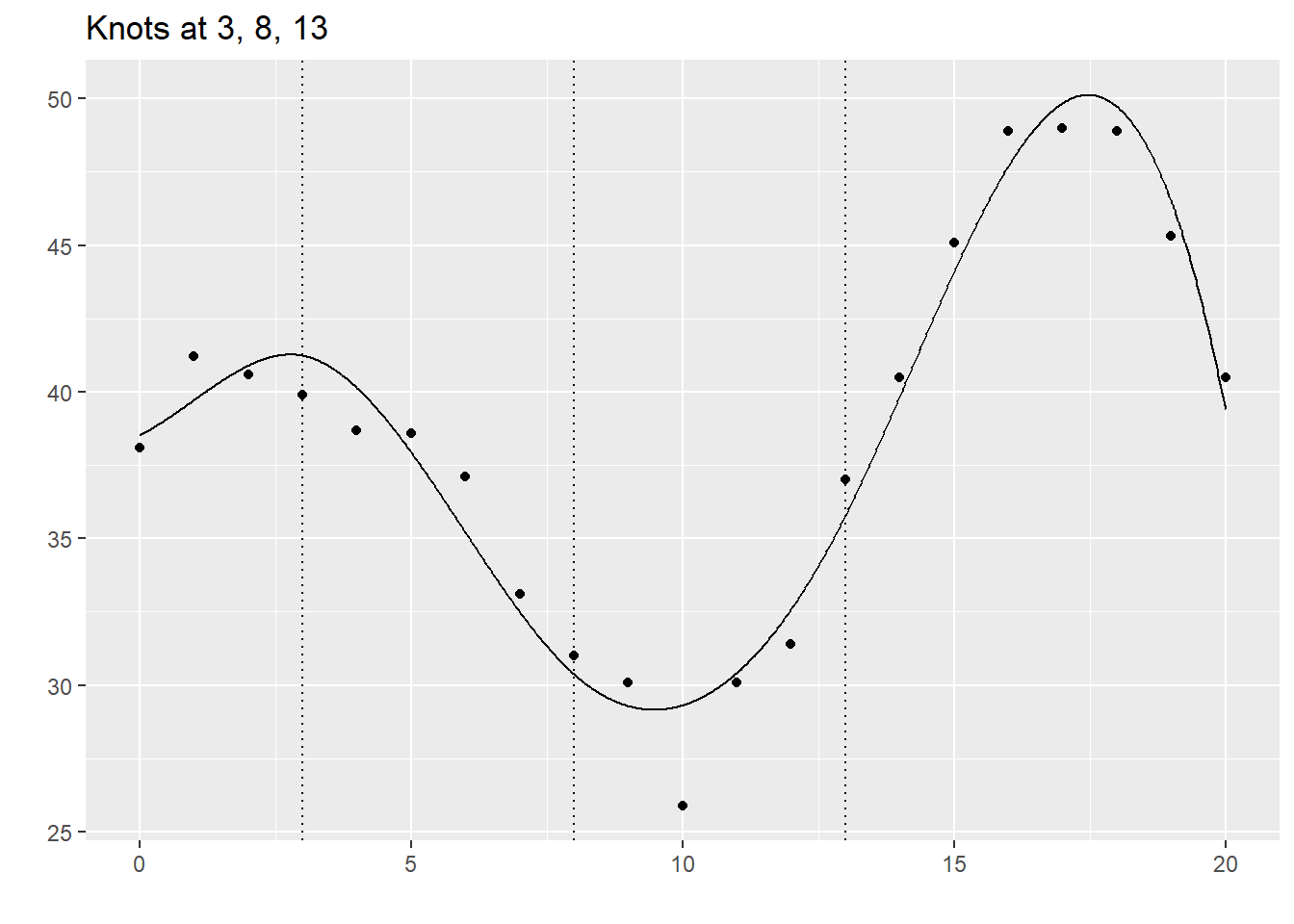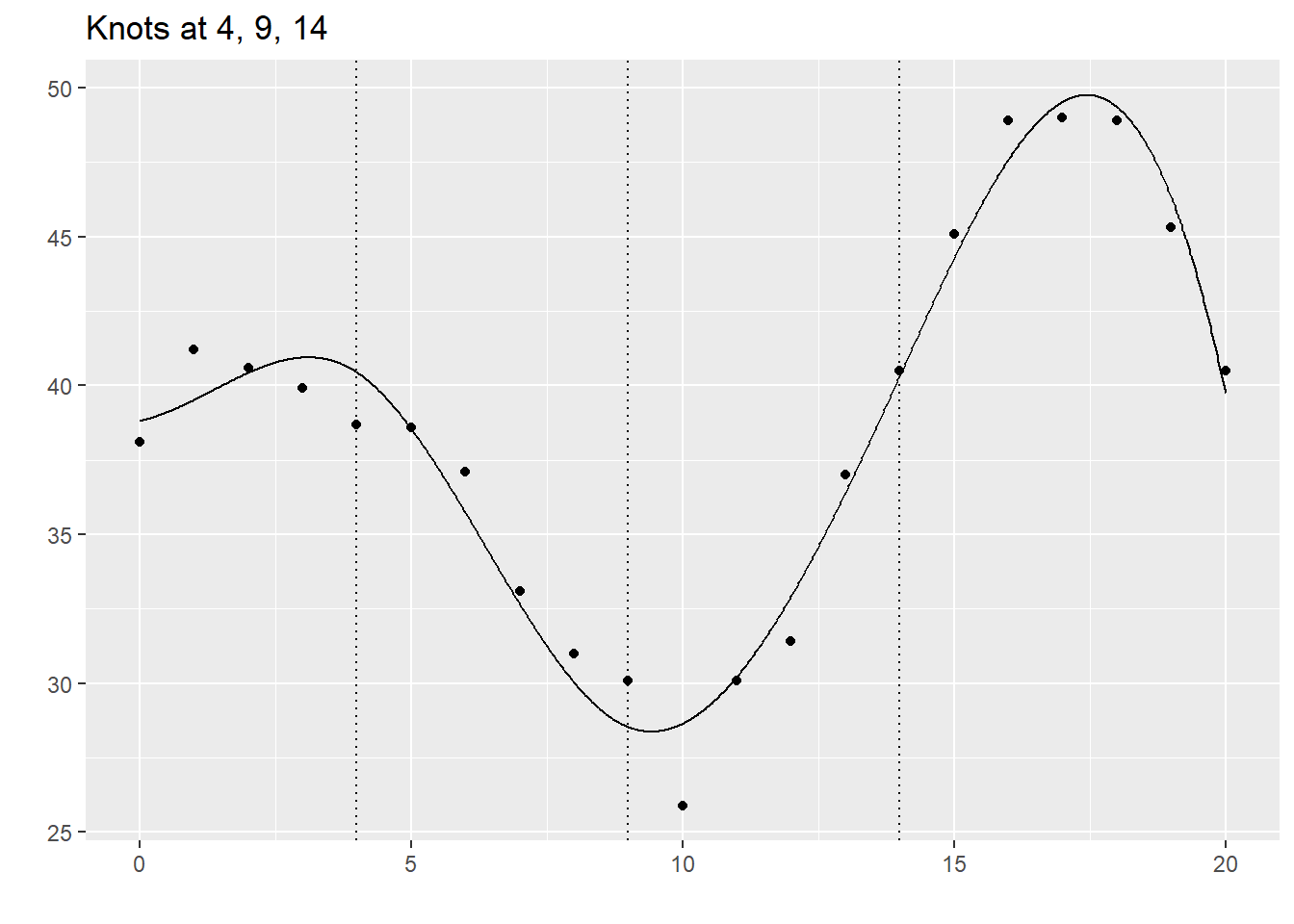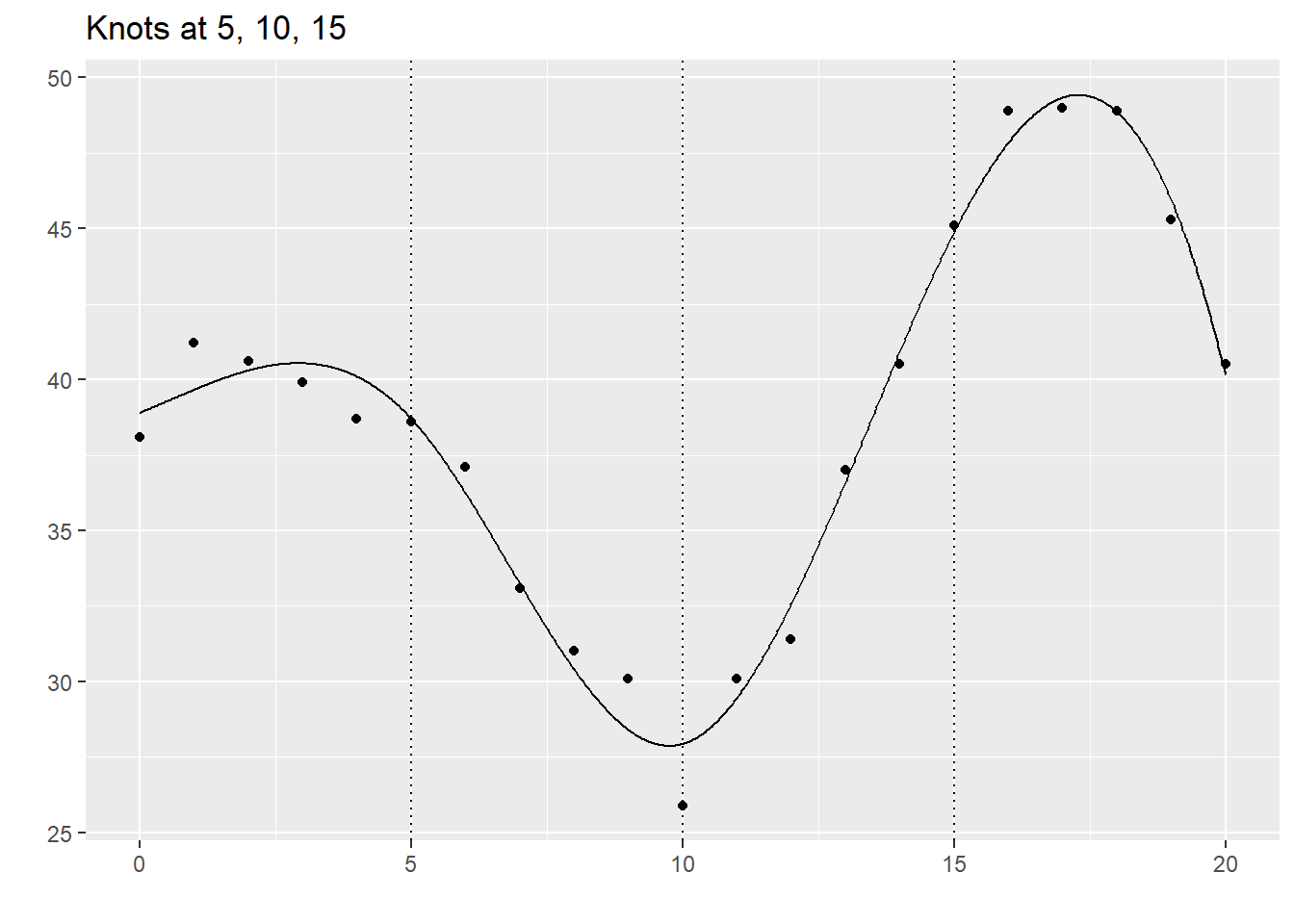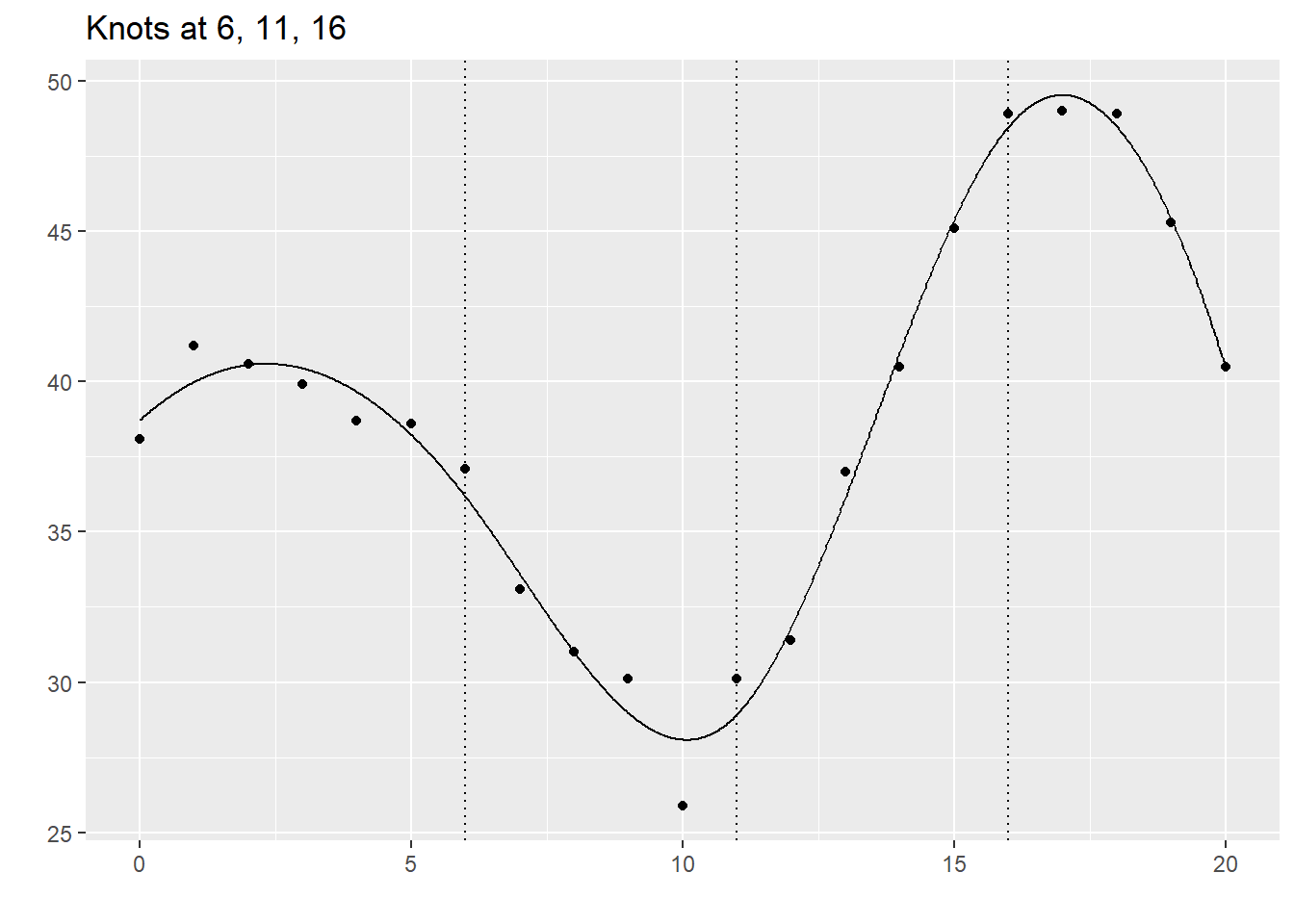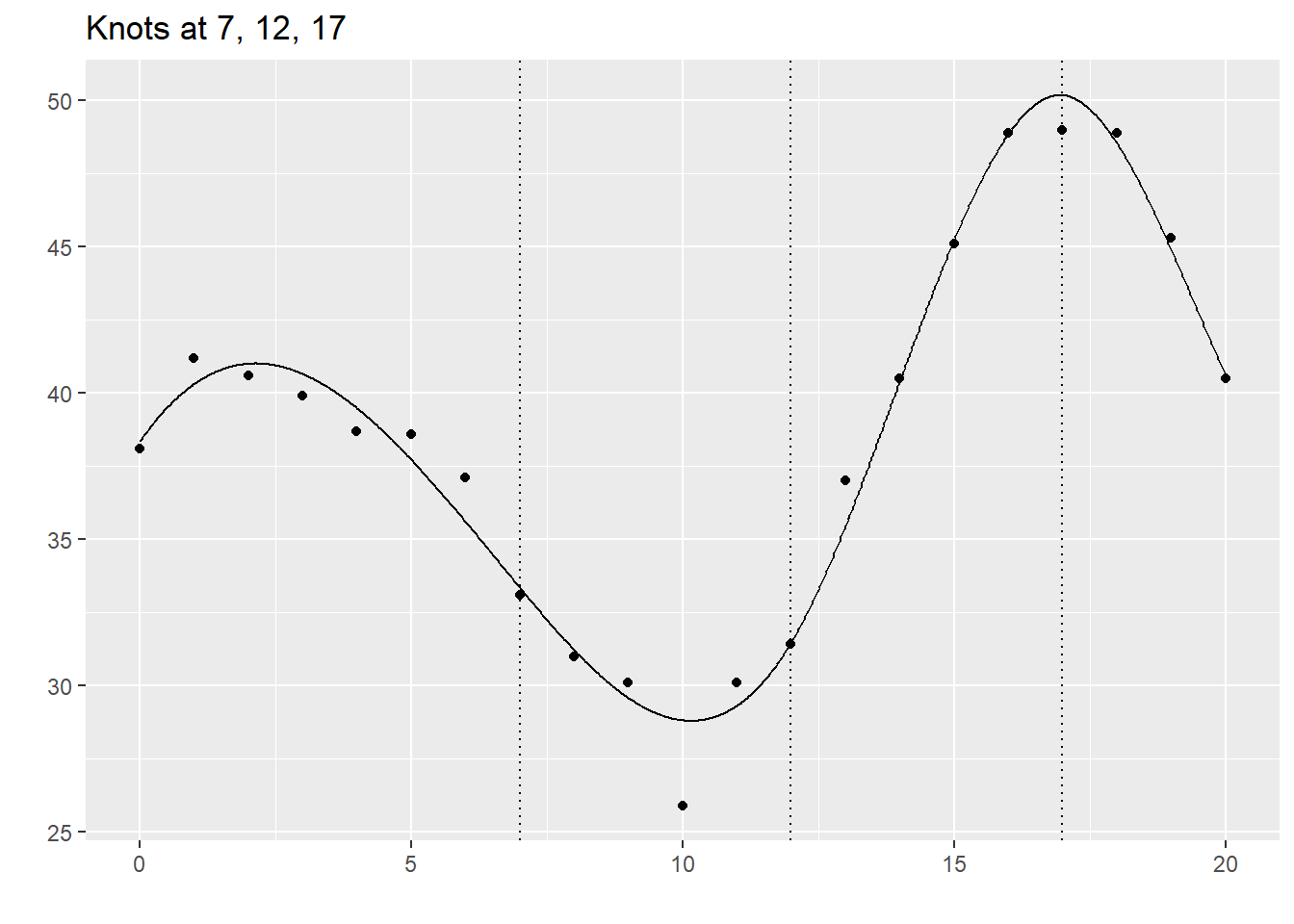There are a few change in the spline functions as the knots move, but the changes are not very large in magnitude.